• 二阶线性微分方程边值问题的MATLAB求解
2021-04-25 14:29:54

2012 年 3 月第 26 卷 第 1 期 阴 山 学 刊 YINSHAN ACADEMIC JOURNAL Mar． 2012 Vo1． 26 No． 1 二阶线性微分方程边值问题的 MATLAB 求解* 云 文 在 ( 包头师范学院 数学科学学院，内蒙古 包头 014030) 摘 要: 本文给出二阶线性微分方程边值问题数值算法的 MATLAB 实现，并举例进行了求解仿真及与解 析解的精度比较。 关键词: 边值问题; 初值问题; MATLAB 中图分类号: O175 文献标识码: A 文章编号:1004 －1869( 2012) 01 －0023 －02 微分方程数值解中，侧重研究初值问题，即已知 x0 对其他时刻状态变量值进行求解的方法。在实际问题中，经常会遇到这样的问题: 已知部分状态在t = 0时刻的值，还知道部分状态在时刻t0 = tf 的值，这类问题即所谓边值问题。而在 MATLAB 语言中边值问题也是 ode45( ) 类函数无法直接求解的一类问题。本文采用将边值问题转化为初值问题的方法，给出二阶线性微分方程的边值问题的计算机求解。 1 边值问题的数学描述 二阶线性微分方程的边值问题的数学描述: ¨ y( x) + p( x)  y( x) + q( x) y( x) = f( x) ( 1) 其中 p( x) 、q( x) 和 f( x) 均为给定函数。假设在区间［a，b］上研究该方程的解，且已知在这两个边界点 上满足边界条件 y( a) = γa，y( b) = γb ( 2) 2 数值求解方法 由于不能直接获得在初始时刻的各个变量的值，因此求解初值问题的通常算法在解边值问题时是不能直接使用的。边值问题数值解法的基本思想是找出能够满足式( 2) 边值的相应初值 y( 0) 和  y( 0) ，然后再利用初值问题算法来求解这一初值问题。该算法也称为打靶算法( shooting method) 。 算法步骤: 求出下面方程初值问题的数值解 y1( b) ¨ y1( x) + p( x)  y1( x) + q( x) y1( x) = 0， y1( a) = 1， y1( a) = 0 求出下面方程初值问题的数值解 y2( b) ¨ y2( x) + p( x)  y2( x) + q( x) y2( x) = 0， y2( a) = 0， y2( a) = 1 求出下面方程初值问题的数值解 yp( b) ¨ yp( x) + p( x)  yp( x) + q( x) yp( x) = 0， yp( a) = 0， yp( a) = 1 若 y2( b) ≠ 0，则计算 m = γb － γay1( b) － yp( b) y2( b) 求出下面方程初值问题的数值解，则 y( x) 即为原边值问题的数值解 ¨ y( x) + p( x)  y( x) + q( x) y( x) = f( x) ， y( a) = γa， y( a) = m 3 上面算法的 MATLAB 实现 求解时应该首先得出对应的一阶微分方程组模型，即设x1 = y，x2 =  y，则得出式( 1) 对应的方程组为:  x1 = x2  x2 = － q( x) x1 － p( x) x2 + f( x{ ) 则上面算法的 MATLAB 实现为 Function ［t，y］ = shooting( f1，f2，tspan，x0f，varargin) 32 * 收稿日期:2011 －11 －04 基金项目: 内蒙古自治区自然科学基金项

更多相关内容
• 第1.2讲 二阶线性微分方程的求解方法 二阶线性微分方程形如 y’’ + P(x) y’ +Q(x) y = f(x)，是二阶微分方程 y’’ =F(x,y,y’)的特殊形式。当f(x) = 0时，称为齐次的，否则称为非齐次的。二阶线性微分方程的力学...

# 第2讲 二阶线性微分方程的求解方法

二阶线性微分方程形如 y’’ + P(x) y’ +Q(x) y = f(x)，是二阶微分方程 y’’ =F(x,y,y’)的特殊形式。当f(x) = 0时，称为齐次的，否则称为非齐次的。二阶线性微分方程的力学背景是加速度，利用牛顿第二定律可以列出二阶线性微分方程。例见同济高数P329。知识点脑图如下：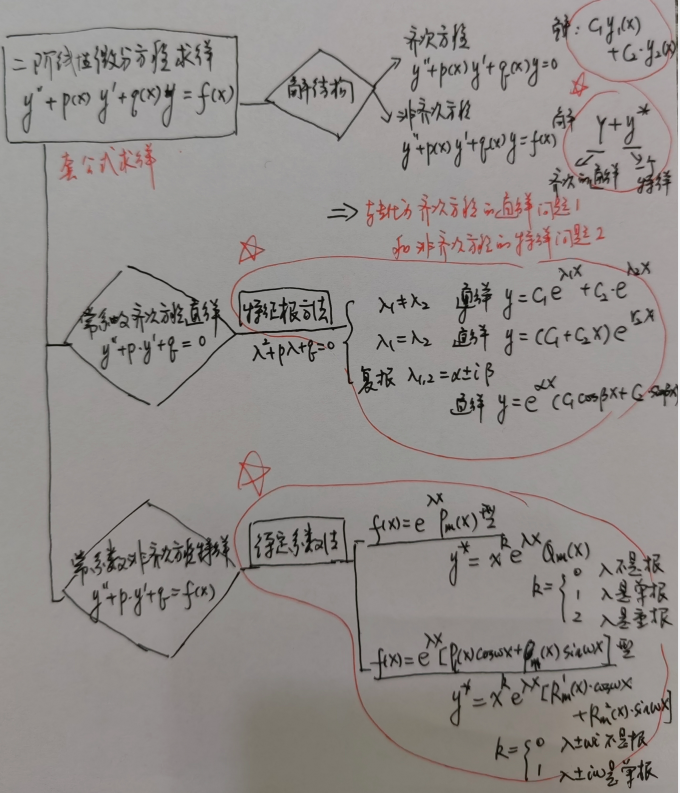# 学习要点

1、和一阶微分方程对应，掌握齐次方程和非齐次方程的解的结构关系
2、牢记二级结论，对定理推导的结果如特征根法求解公式。否则做题时重新推导速度太慢
3、学习和练习的要点就是典型模型识别和套公式的转化化归。因为很多解是采用构造法得出的，能套上合适的模型就是一种能力。不要看不起套公式的方法。

# 一、解结构

## 1、二阶齐次方程的通解C1y1(x)+C2y2(x)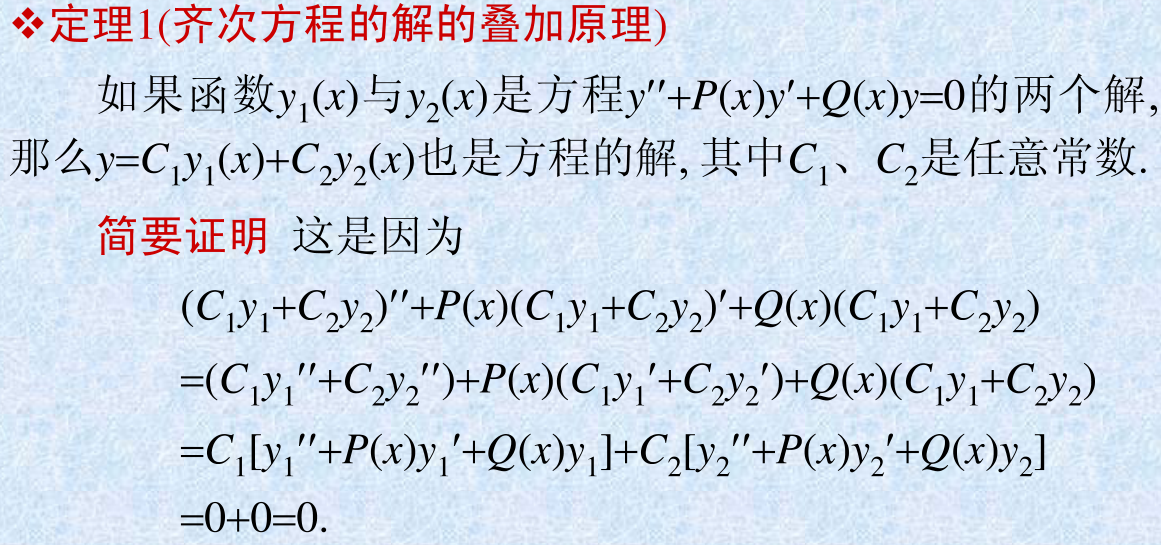当y1(x)和y2(x)是线性无关的，y= C1 y1(x) + C2 y2(x) 就是齐次微分方程的通解。注意，两个函数只要不是倍数关系，就是线性无关的。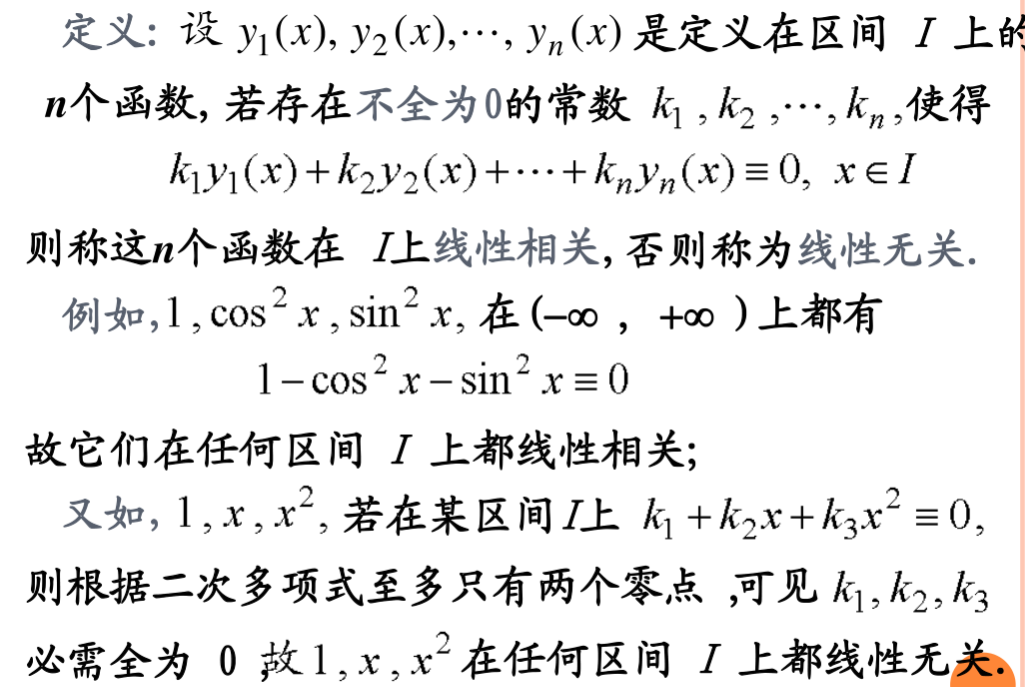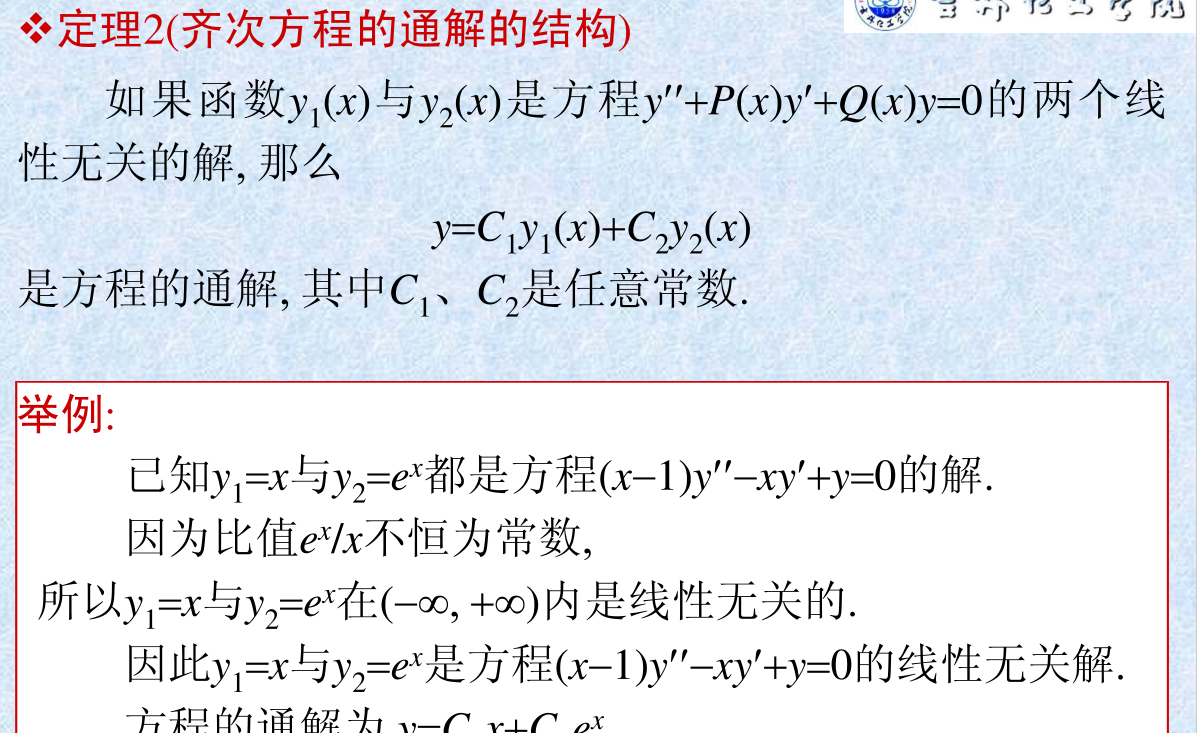## 2、二阶非齐次方程的通解 Y + y*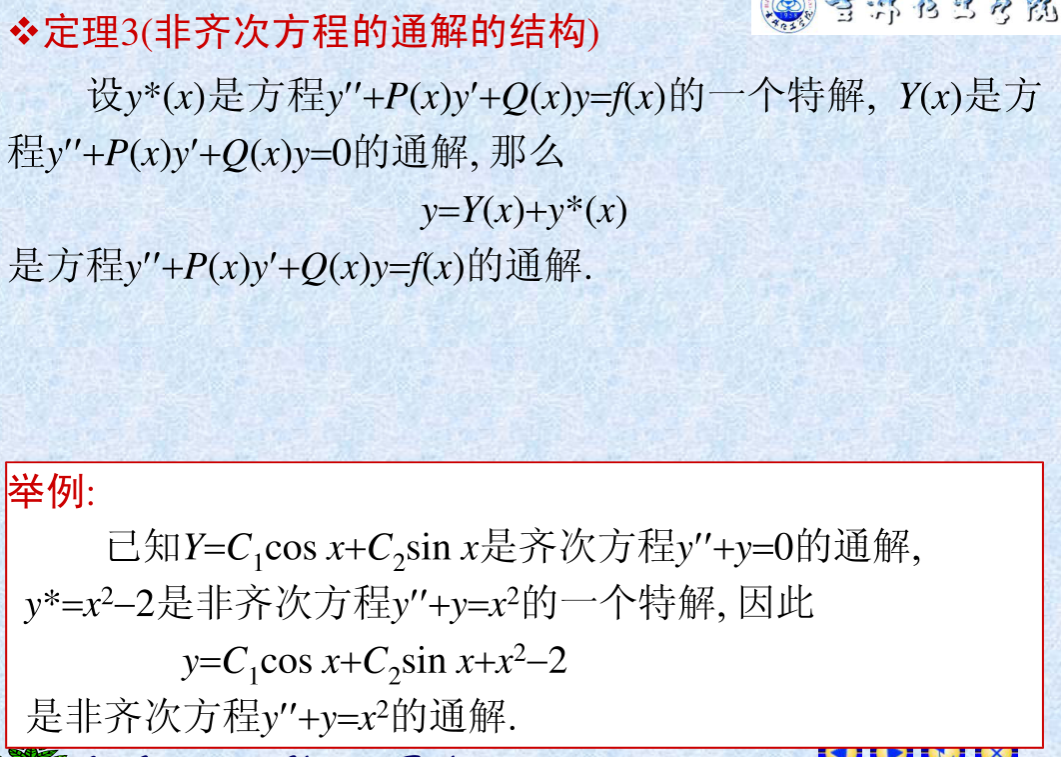证明比较简单，可见同济高数P333。
可以看出，二阶线性微分方程的求解问题转化为两个问题：一是齐次方程的通解求法；二是非齐次方程的特解求法。其中，对常系数微分方程有通解公式，对一般的非齐次方程有常数变易求解方法，具体方法见同济高数P336，具体看是否有教学考核要求。

# 二、常系数齐次线性微分方程通解的特征根解法

一般的变系数齐次微分方程的通解是难以求出的，但对于常系数齐次微分方程 y’’ + py’ +qy =0 ,可以采用特征根方法，给出通解形式。实际计算中可以绕过推导过程，直接套用公式。
根据第一部分齐次方程的通解结构，只要找到两个线性无关的解y1(x)和y2(x),就可以根据 C1y1(x)+C2y2(x)写出通解。因此关键是构造出两个这样的解。采用了erx进行构造。这种构造方法称为特征根方法 。

## 1、特征根求解公式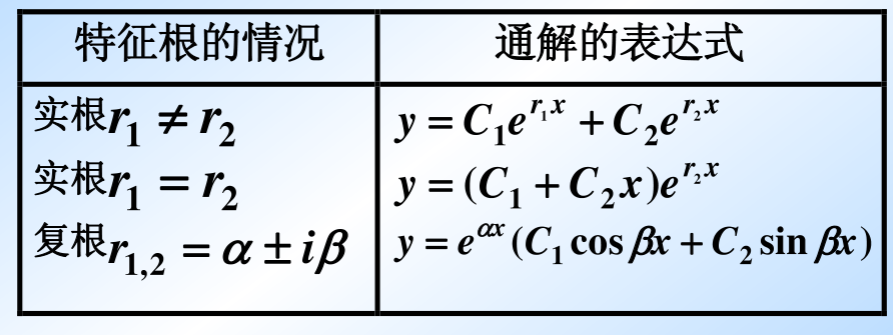在实际求解时可以套用公式，下面简要给出推导过程。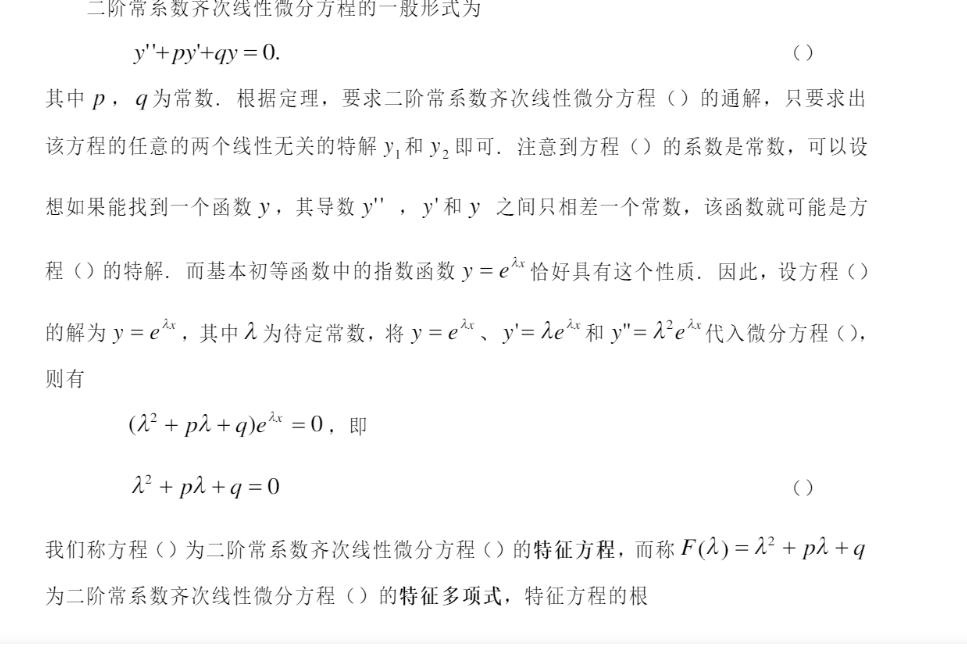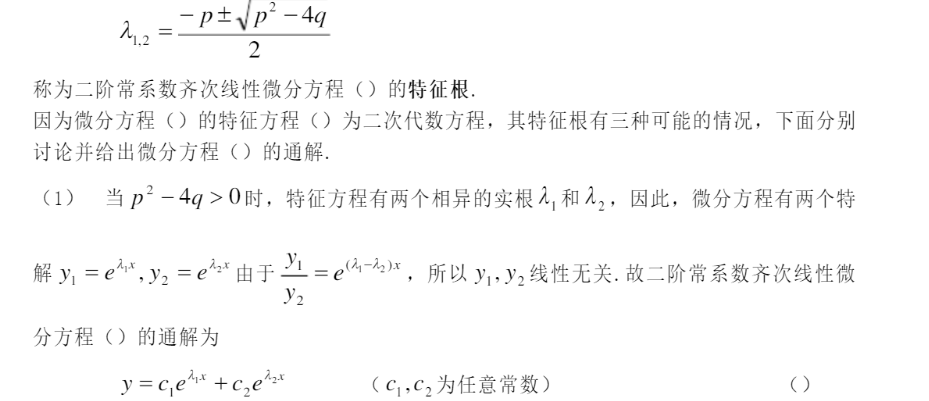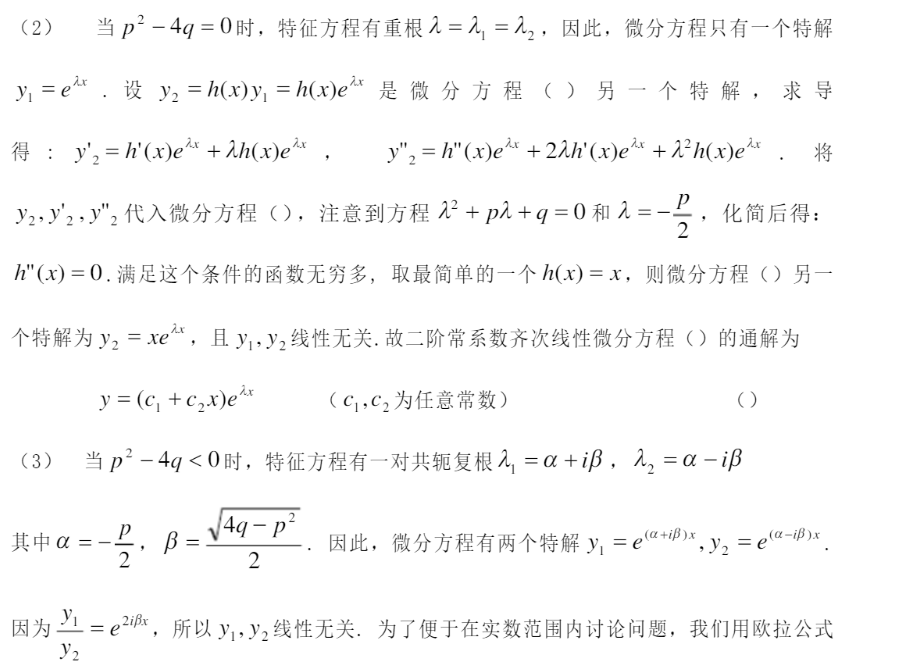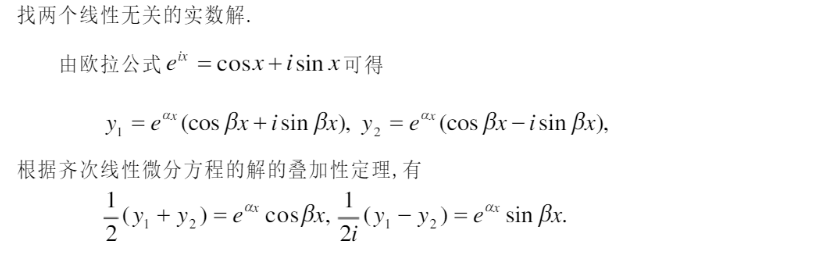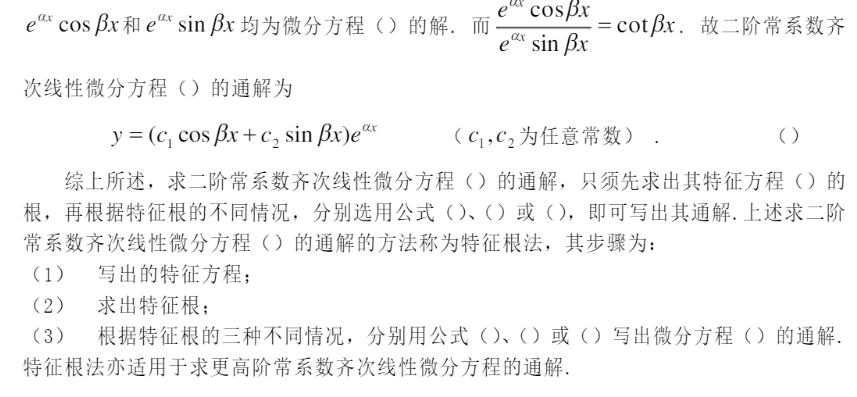## 2、几个求解例子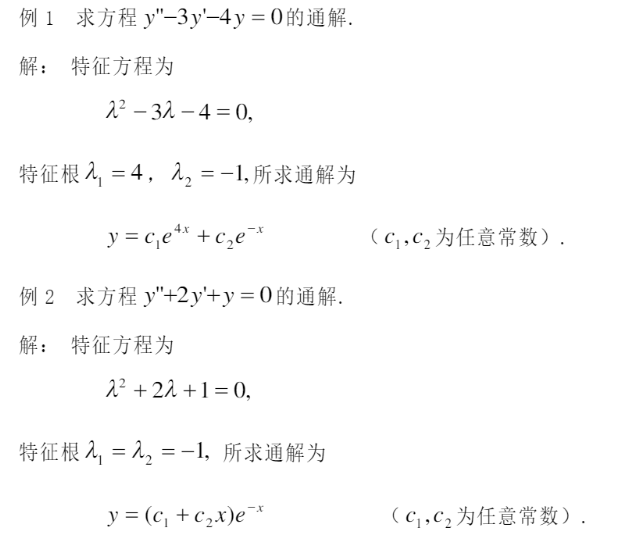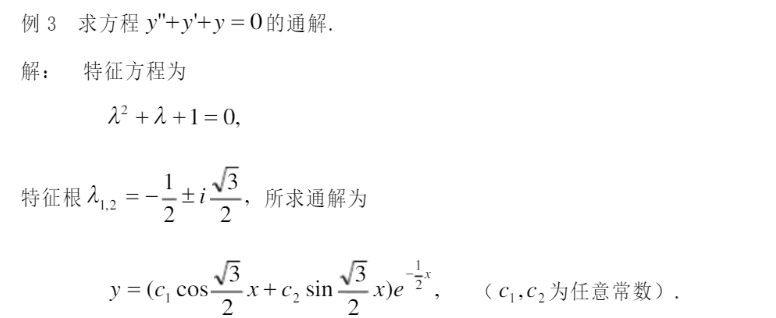## 3、变形问题：从特解反求微分方程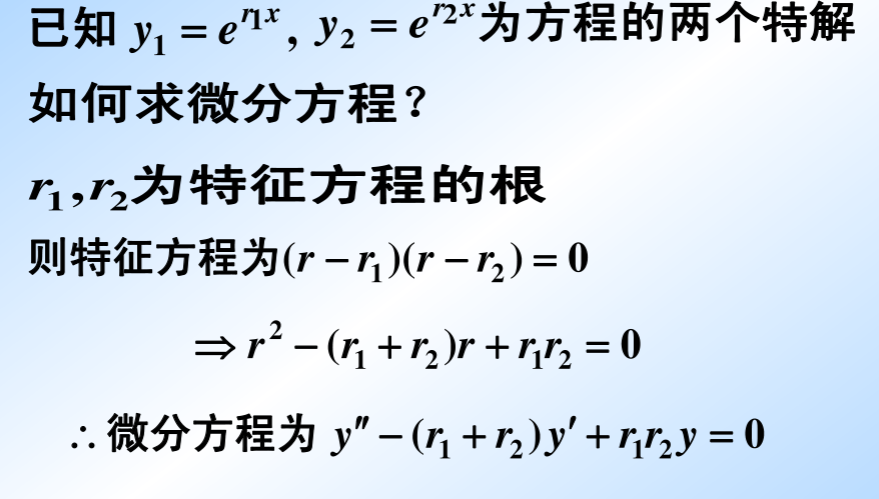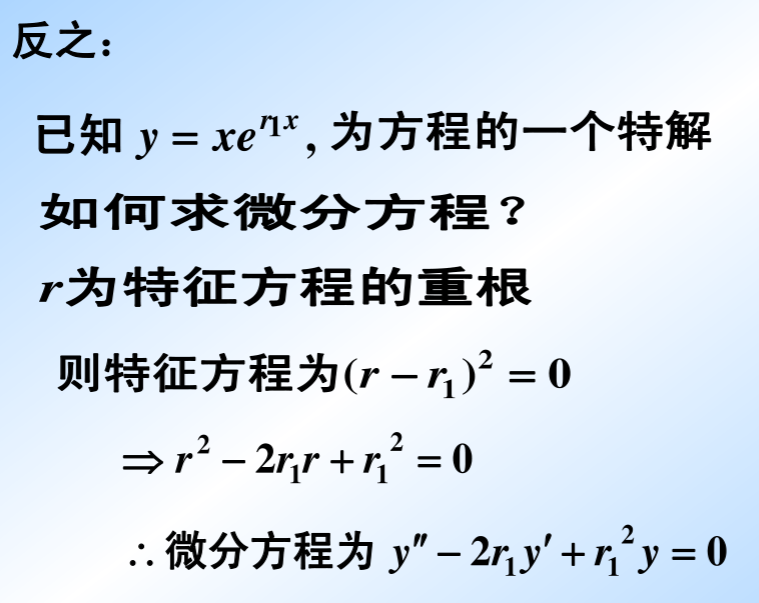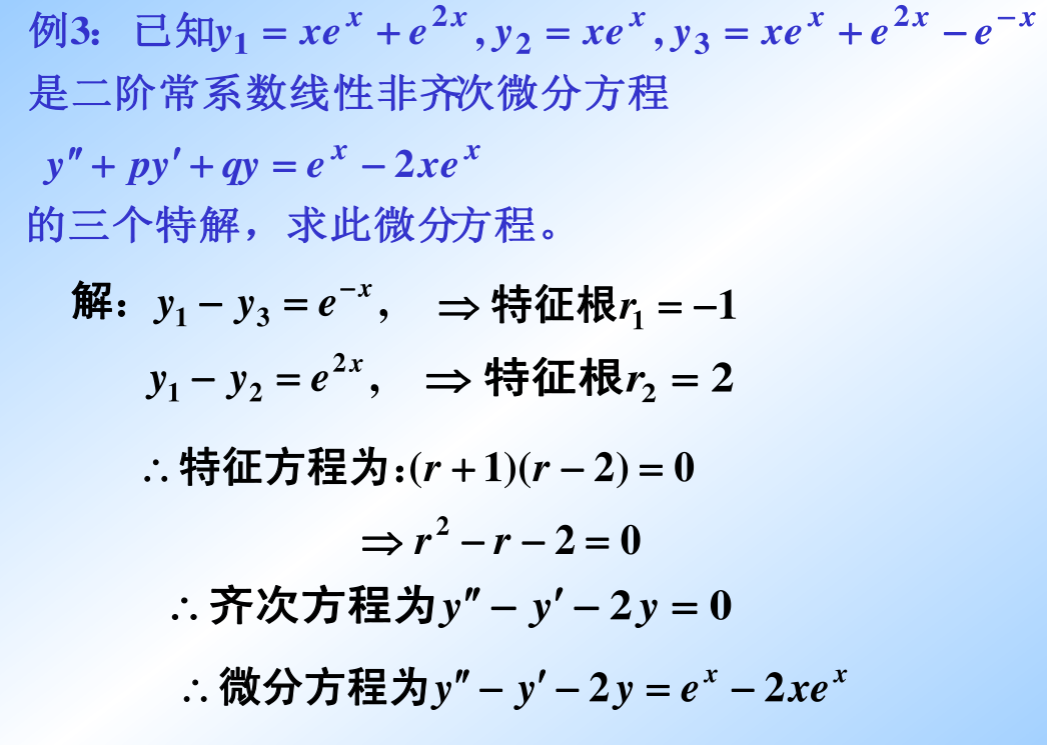注意特解的构造性，利用了齐次方程特解线性组合也是特解的性质，目的是为了构造出erx的特解。

# 三、常系数非齐次线性微分方程特解的待定系数法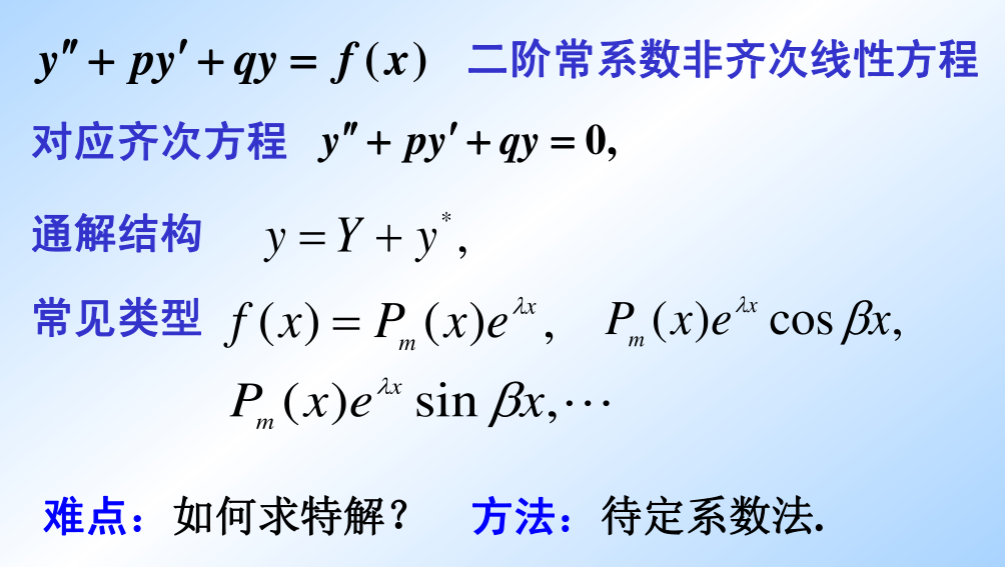非齐次方程的解结构为 齐次方程通解加上非齐次的一个特解，而利用特征根法已经解决了通解求解问题，下面将针对几种特殊的f(x)给出特解求解方法，其本质是构造法给出特解，其特点是不用积分就能求出，称为待定系数法。

## 1、f(x) = eaxPm(x)型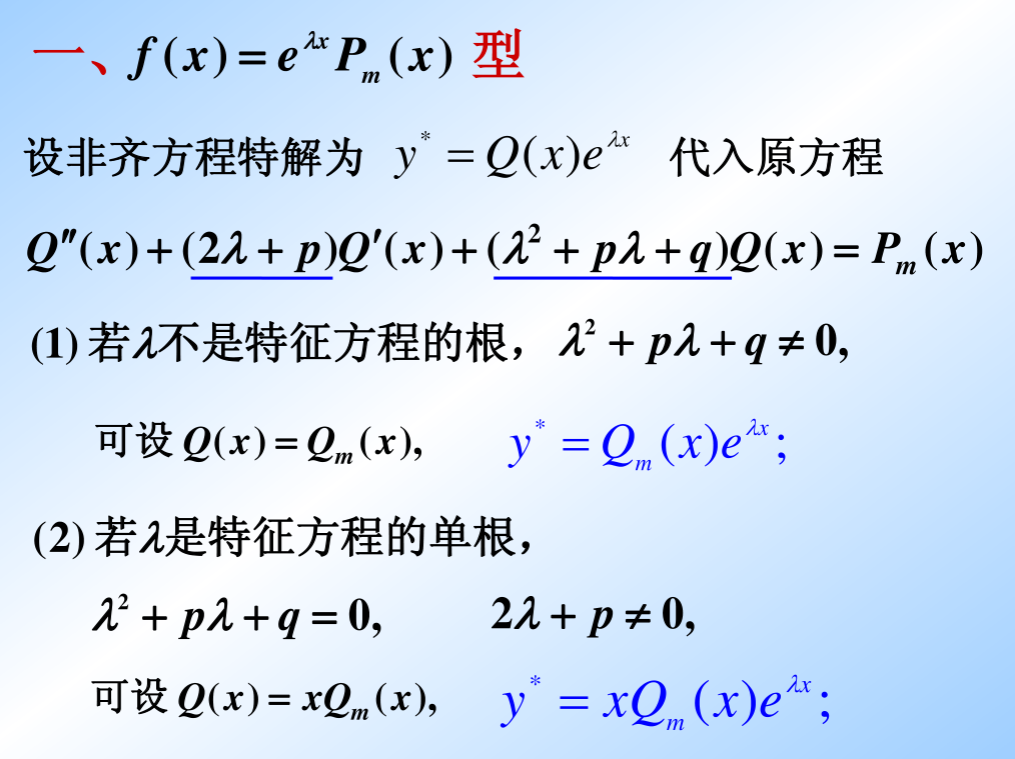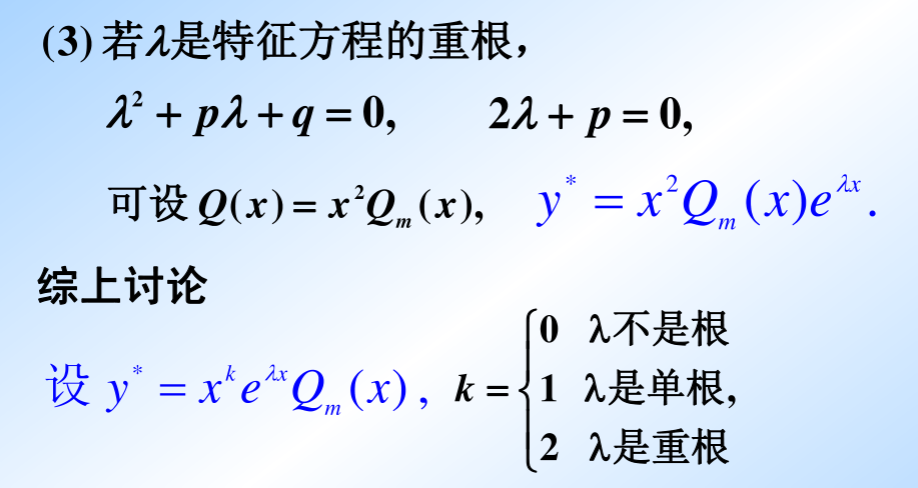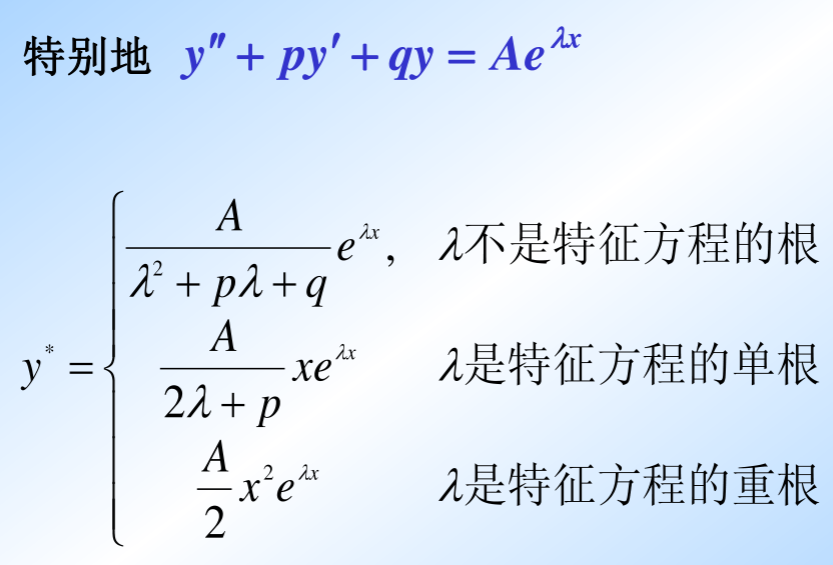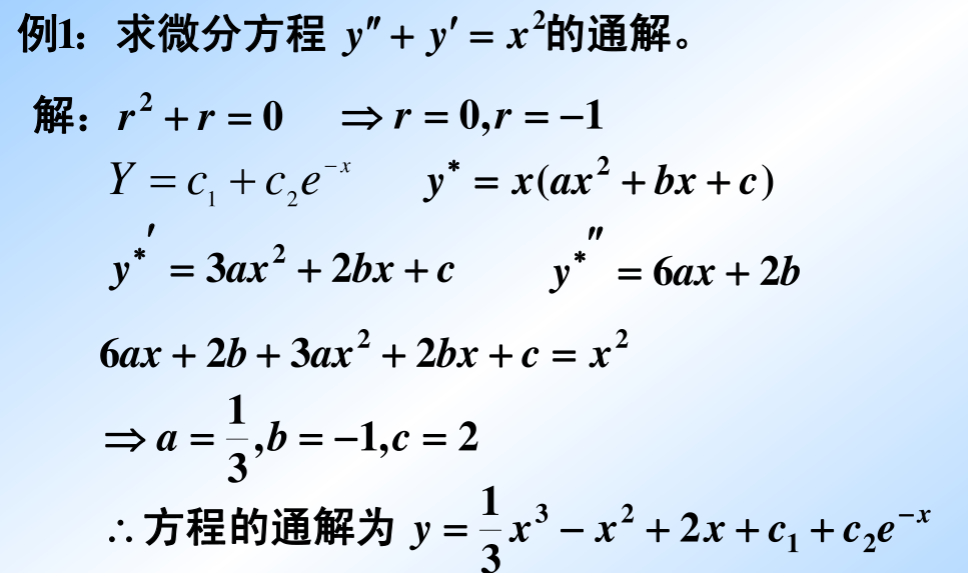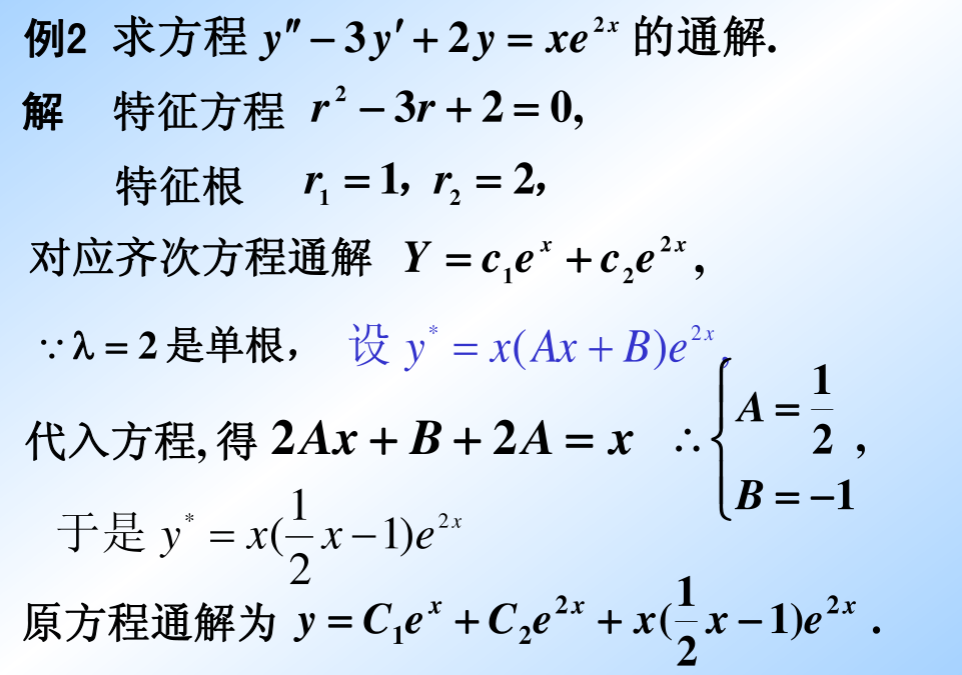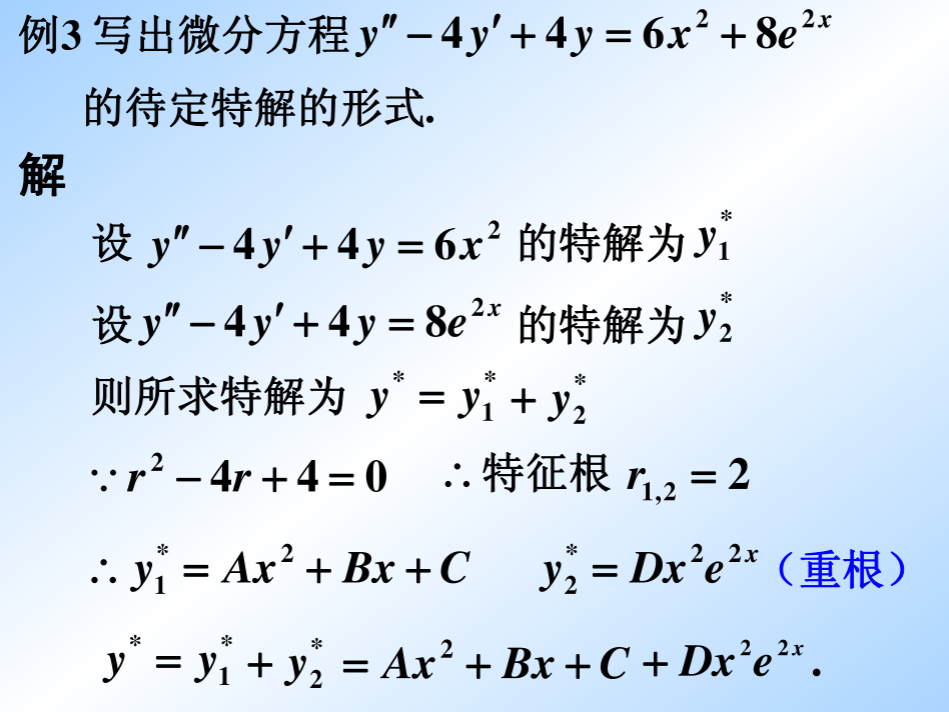## 2、f(x) = eax[Pl(x) coswx + Qn(x) sinwx]型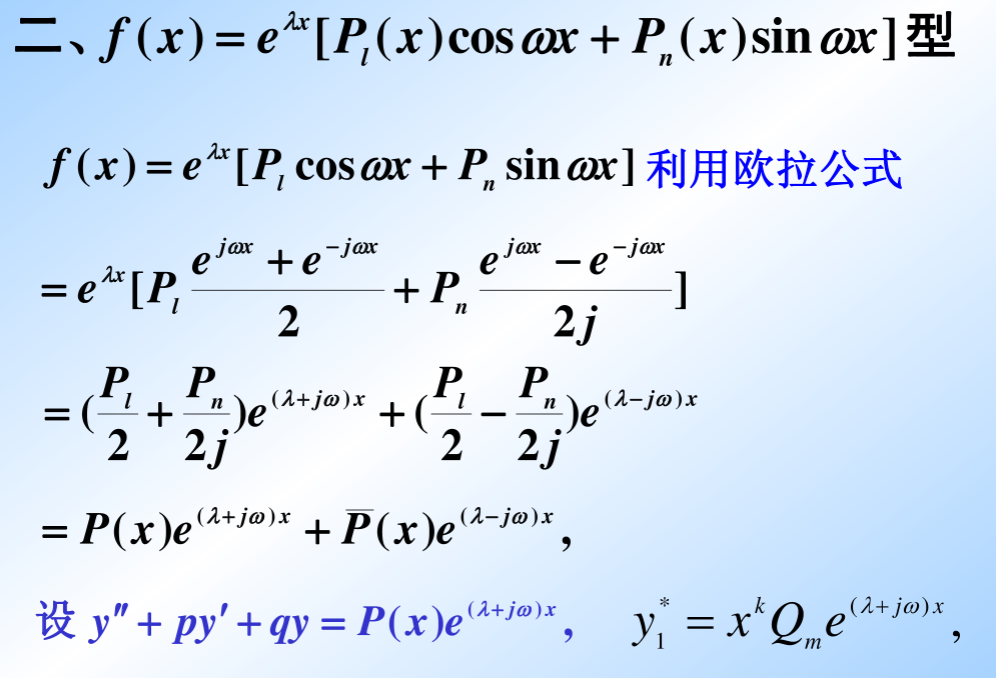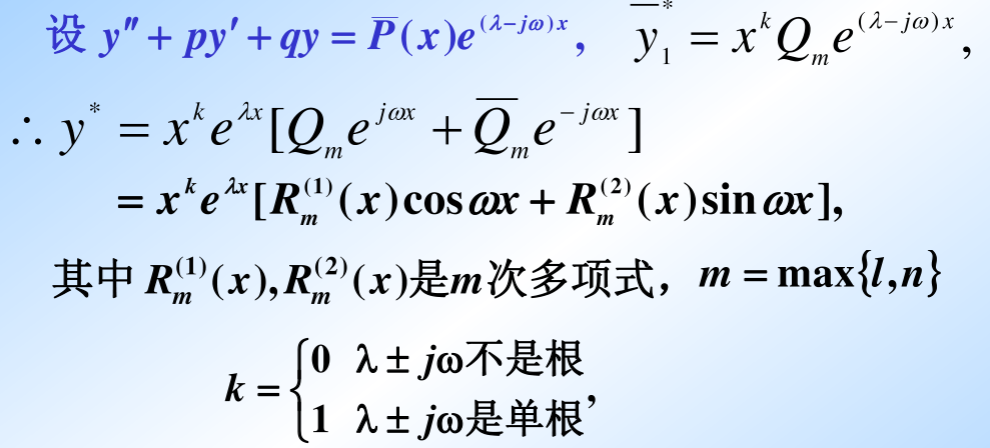待定系数法的本质就是构造出了特解，只要确定系数就可以，所以牢记二级结论的公式定理很重要。举几个例子加强理解。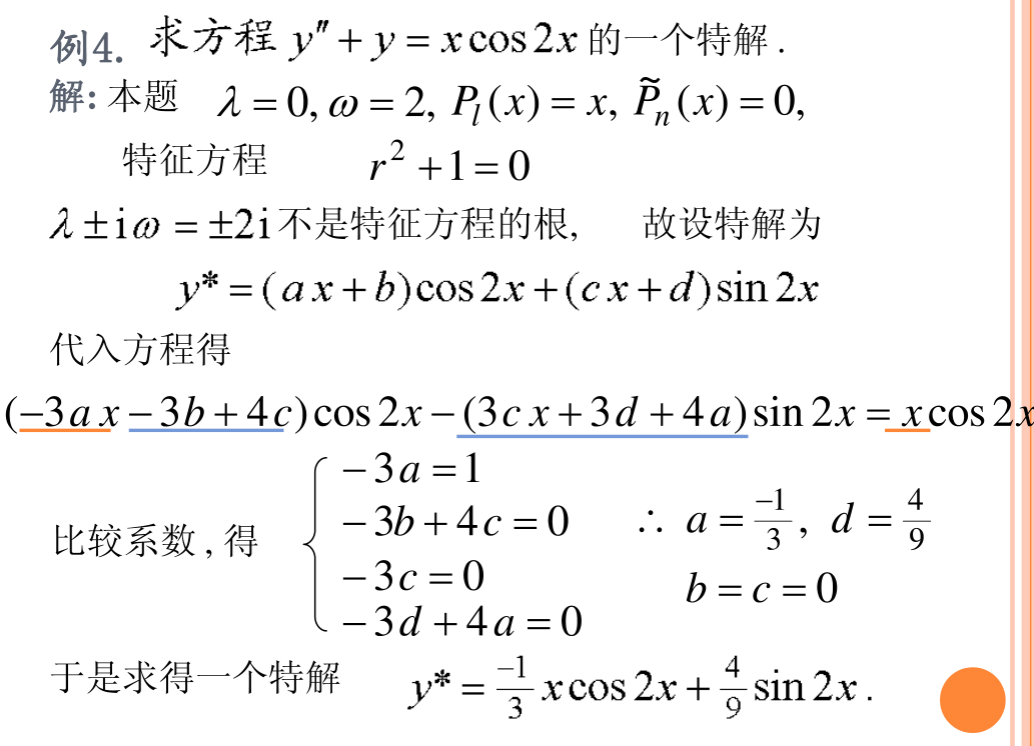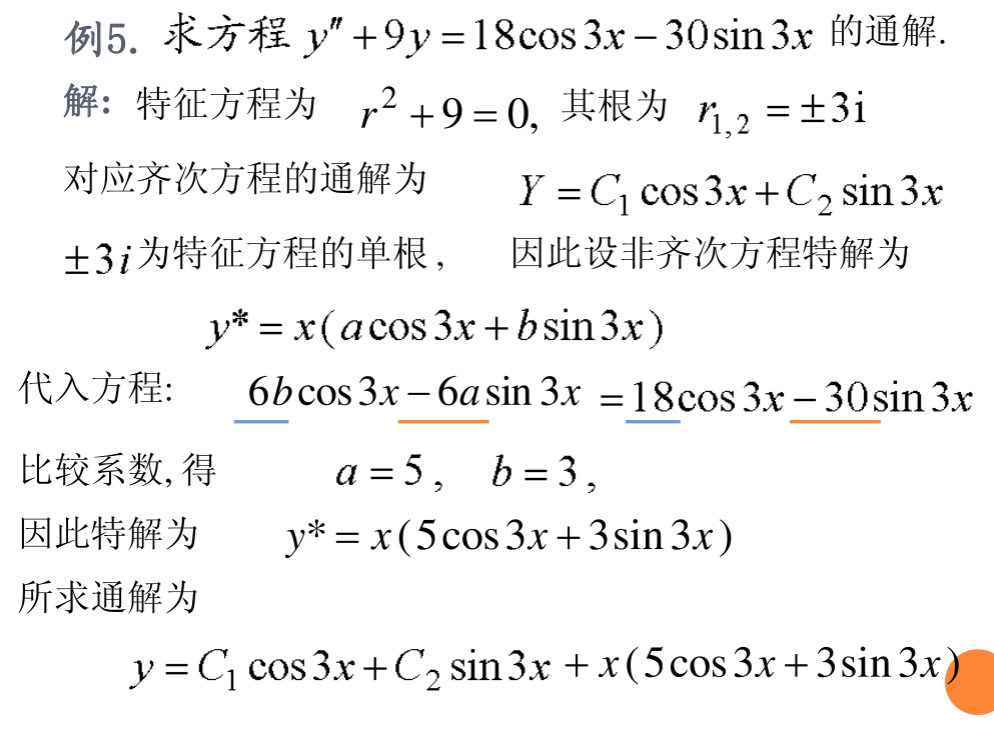如果对复数形式比较熟悉，可以更加简洁一点的过程。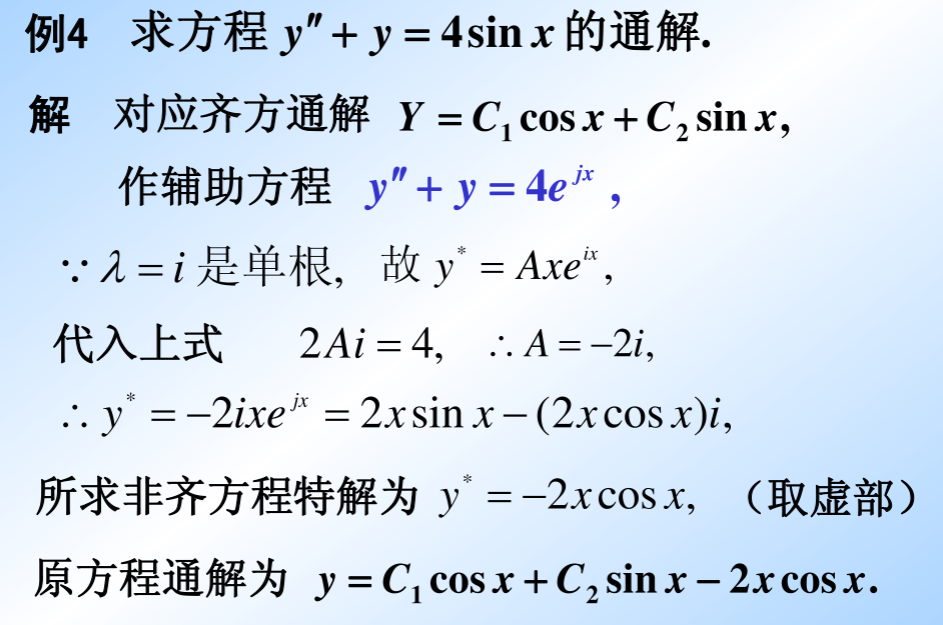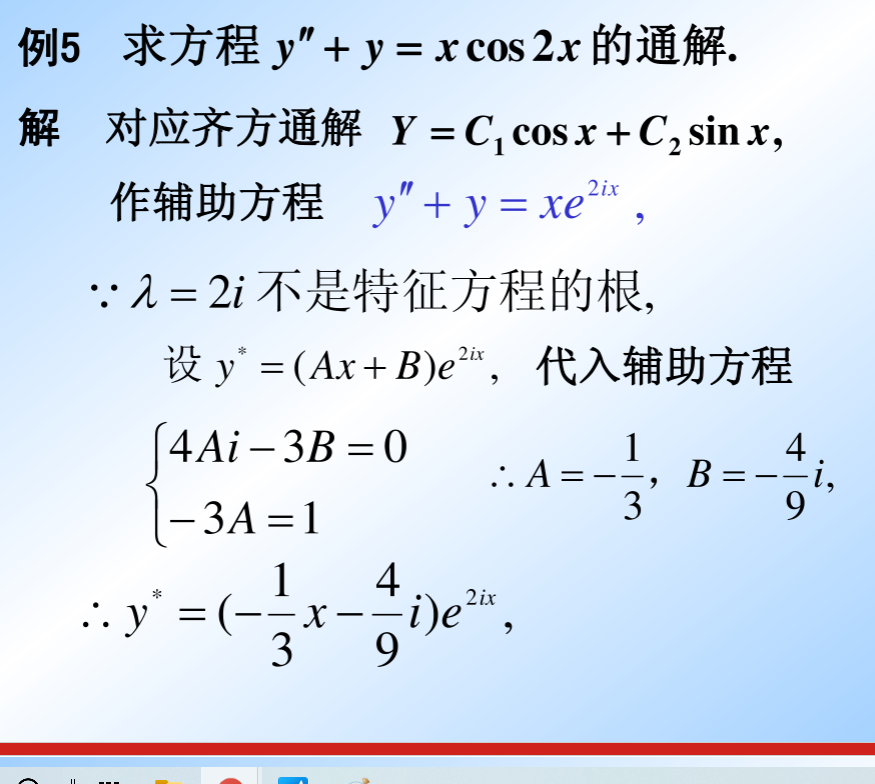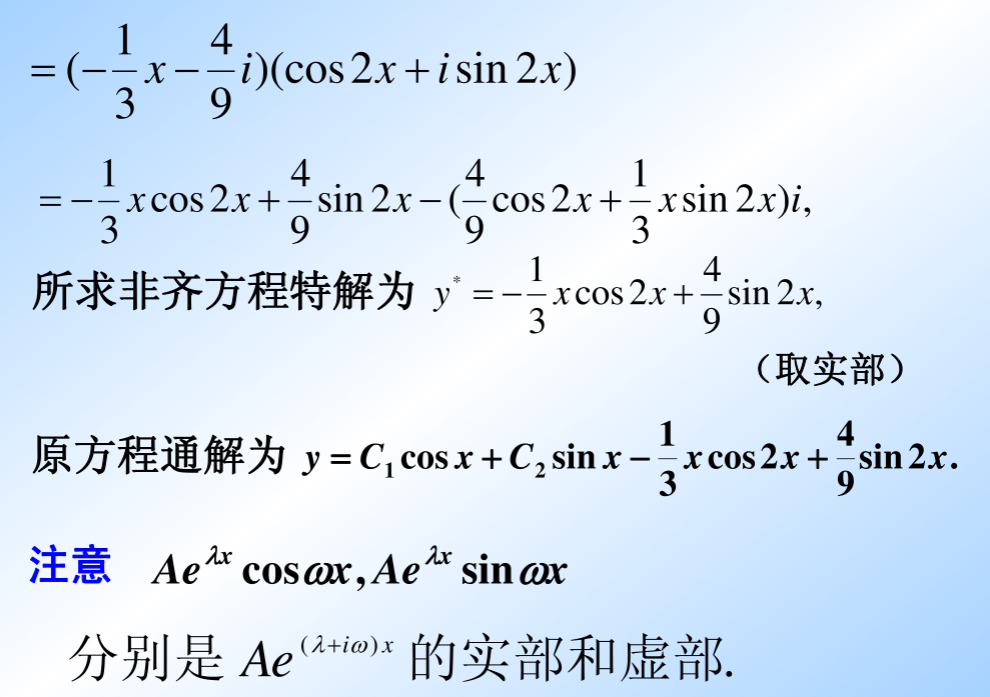展开全文矩阵
•文档
•文档
•文档
•文档
• 一阶二阶常系数微分方程的形式以及通解的解法

最近各学科上都要用到微分方程的知识，发现自己微分方程差不多忘光了，而且由于书没有带回家，所以就想写个博客留着自己复习用。

先说一阶常微分方程：

有以下几种：

1.可分离变量型微分方程

2.一阶齐次线性微分方程

3.一阶非齐次线性微分方程

4.伯努利方程

他们彼此之间的联系是比较明显的，且看我对他们解法的总结。

可分离变量型微分方程：

#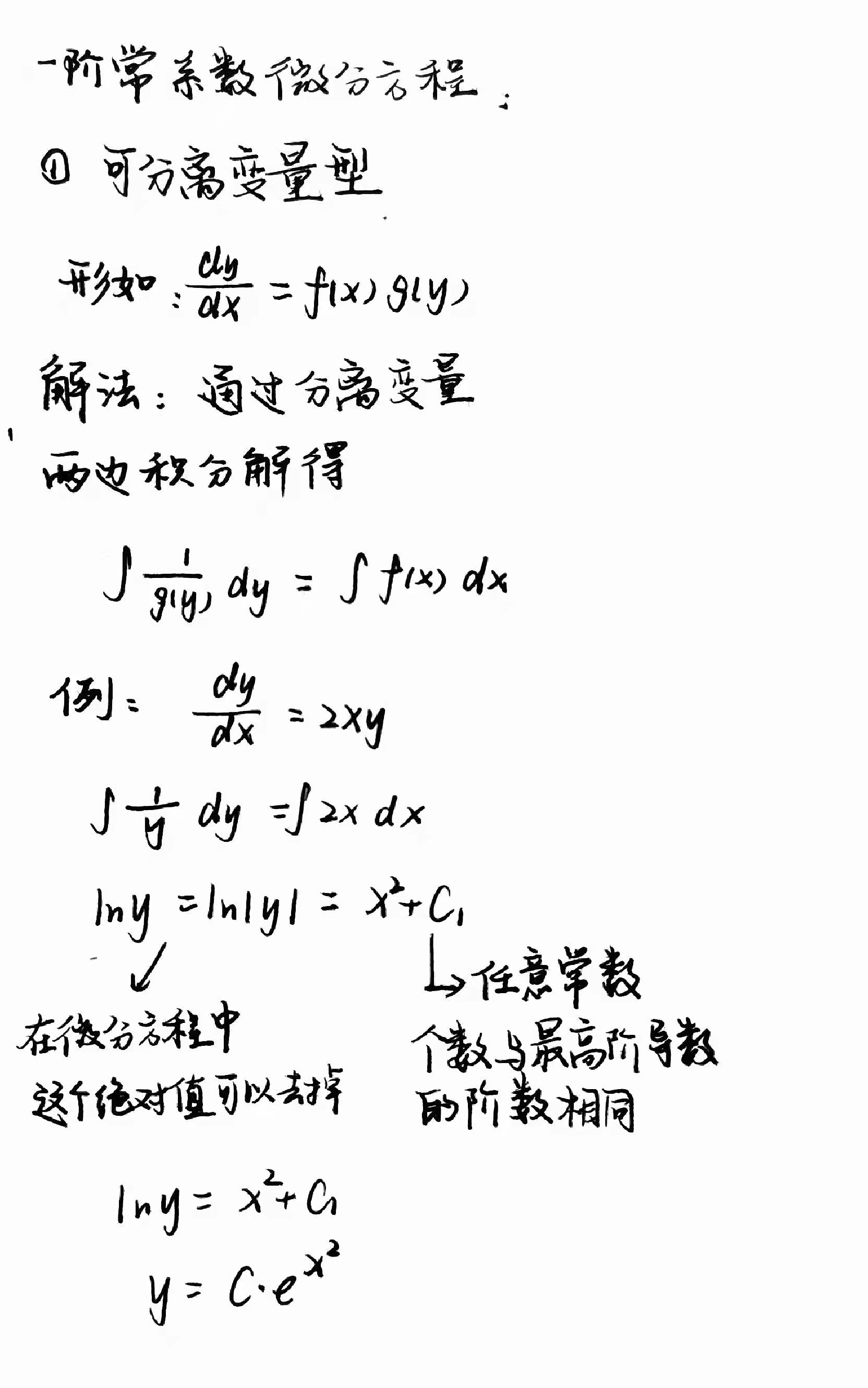一阶齐次线性微分方程（本质上是可分离变量的微分方程）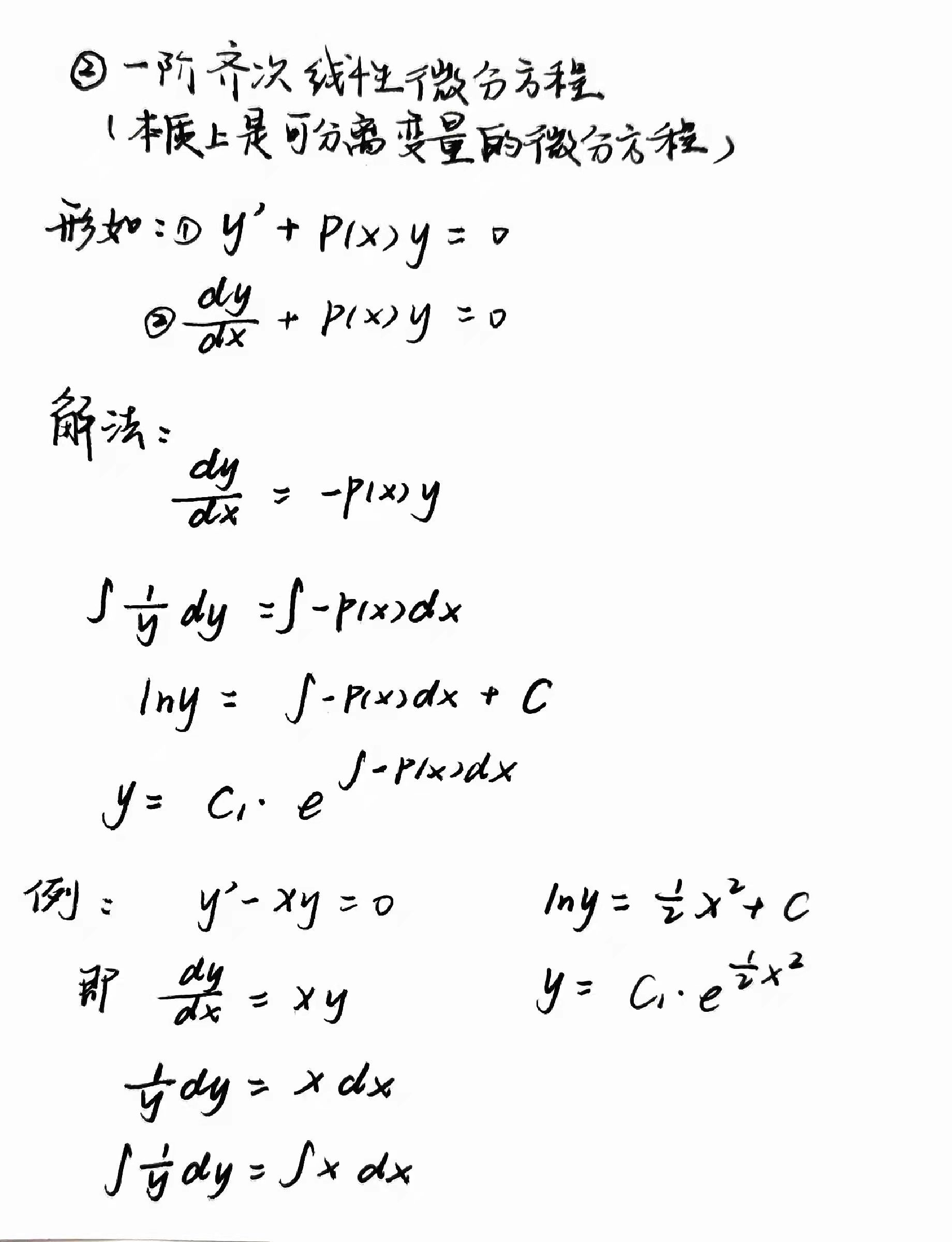一阶非齐次线性微分方程

这里需要用到所谓的常数变易法：即是把一阶非齐次线性微分方程右边不为0的项变为0，这样就可以按一阶齐次线性微分方程解得此时的y，再把y的表达式中的任意常数变为关于x的方程（c(x))，再将此时y的表达式代回微分方程，反求出关于x的方程（c(x));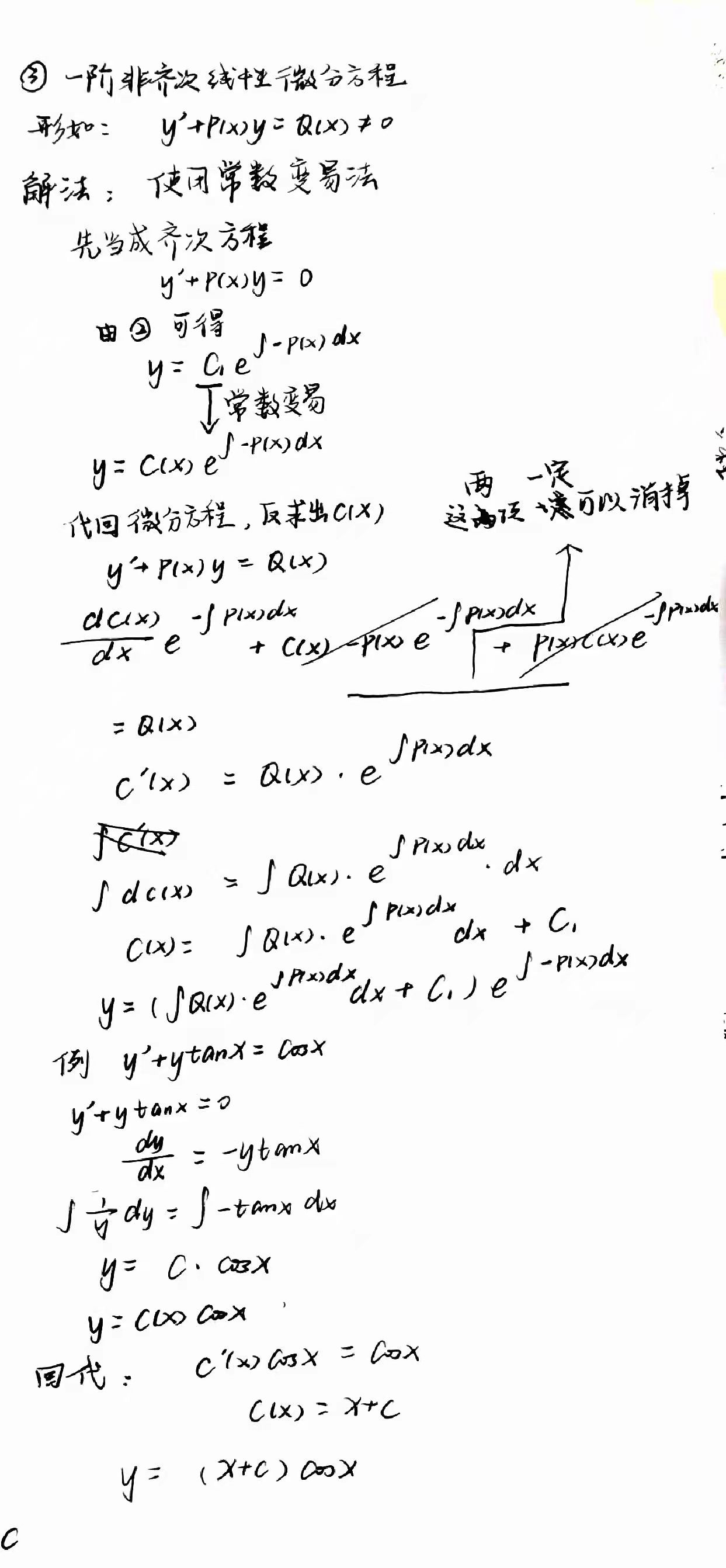伯努利方程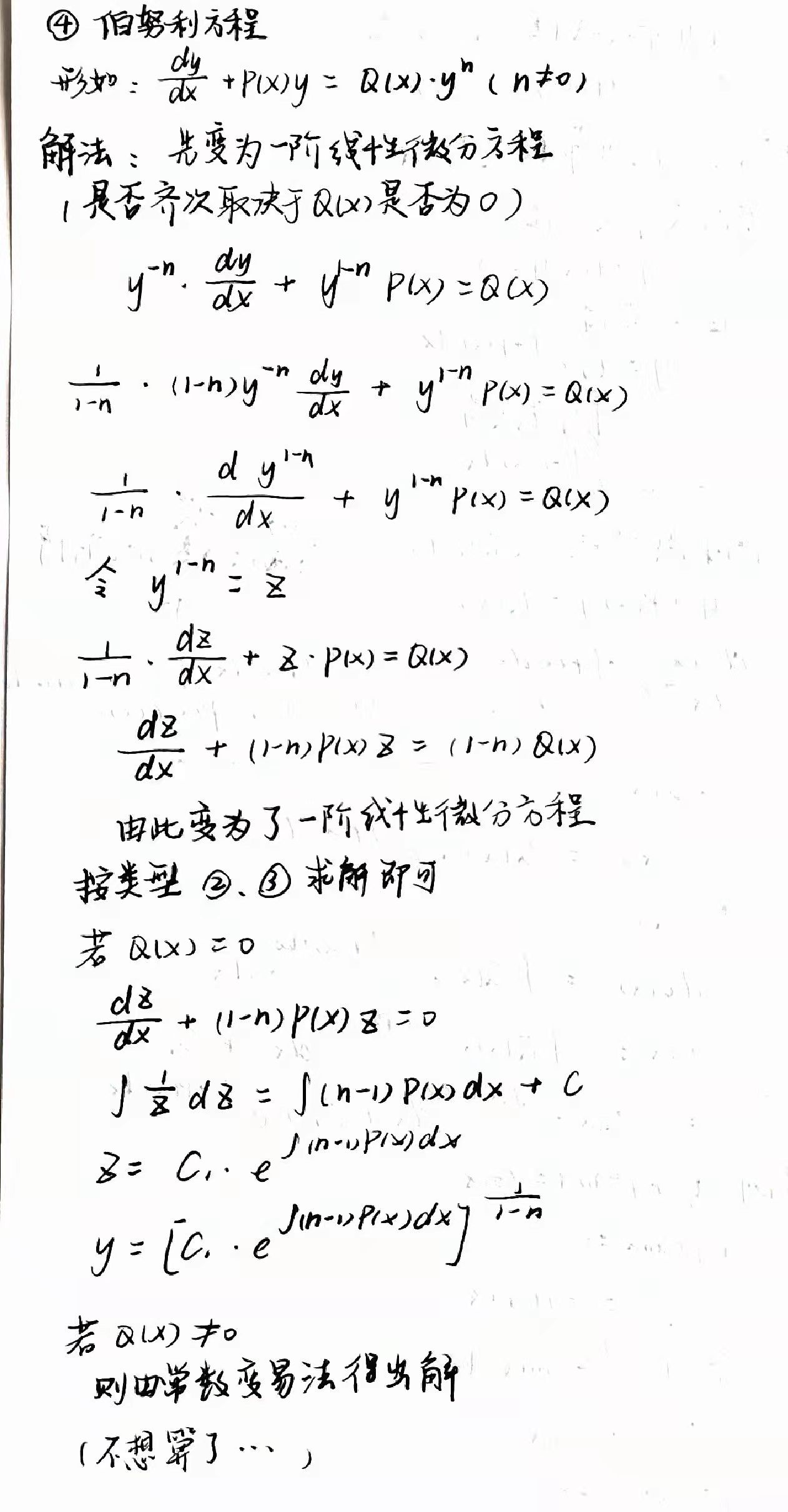接下来是高阶微分方程：

其实也就是可降阶微分方程和二阶微分方程；

可降阶微分方程：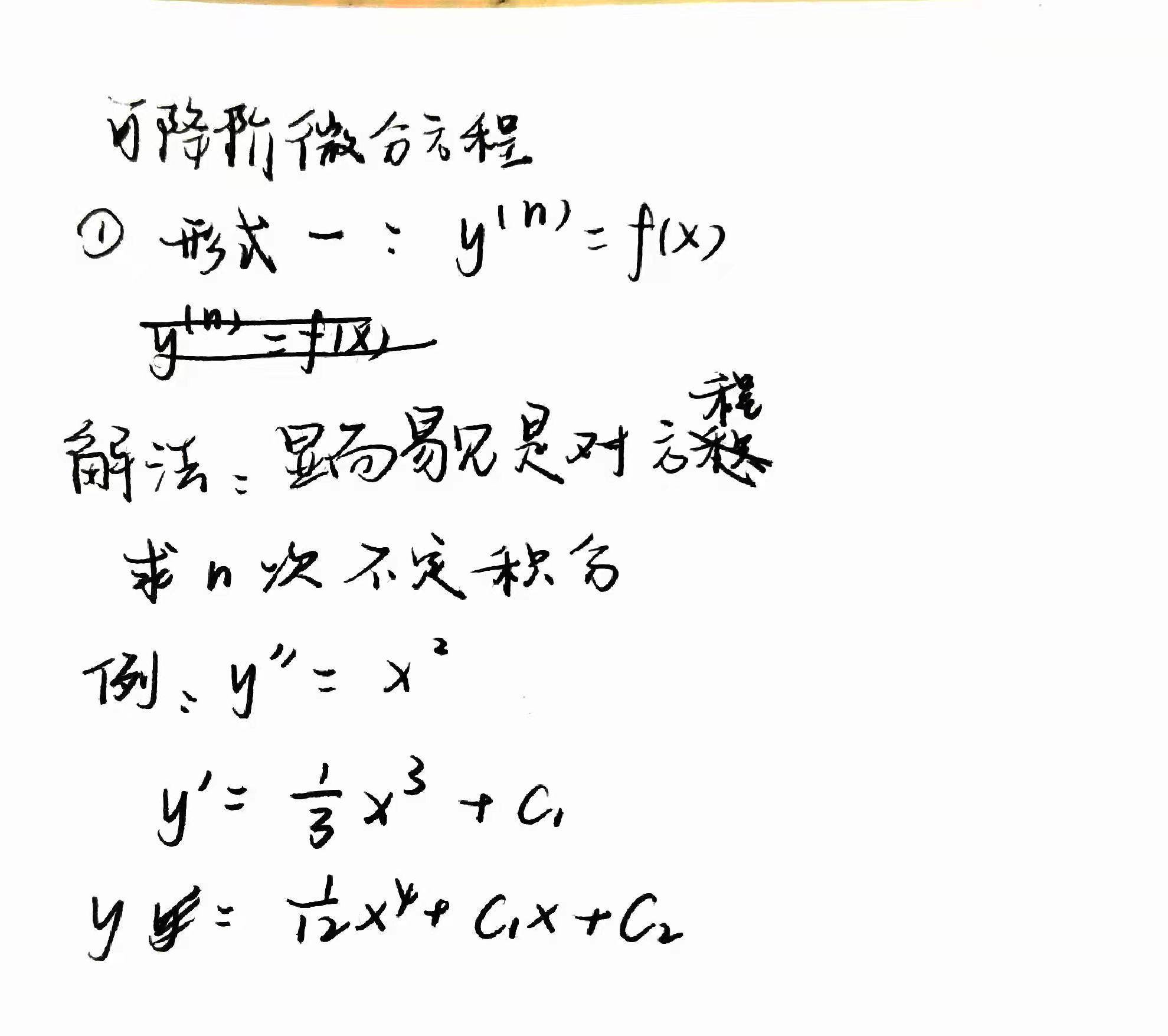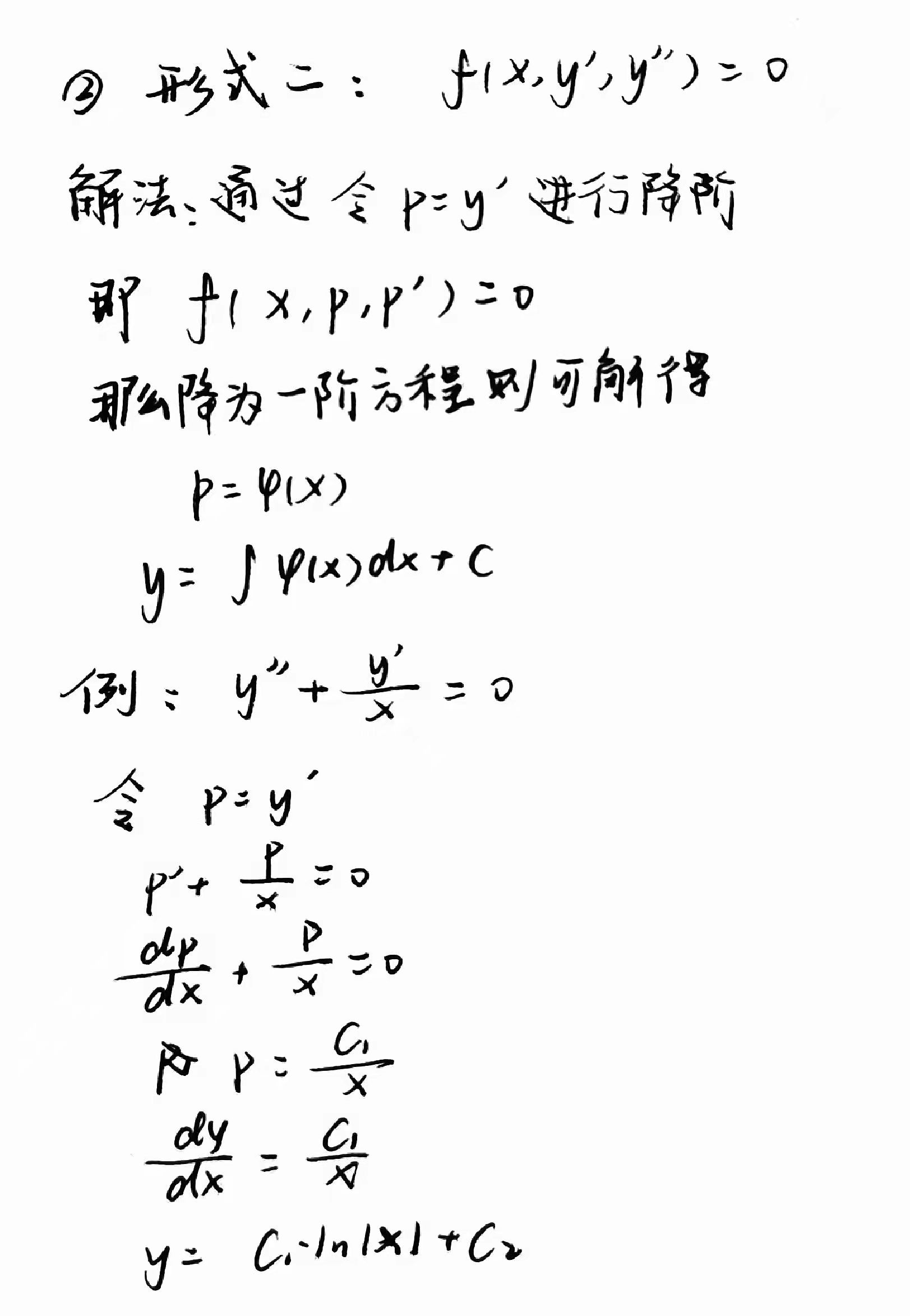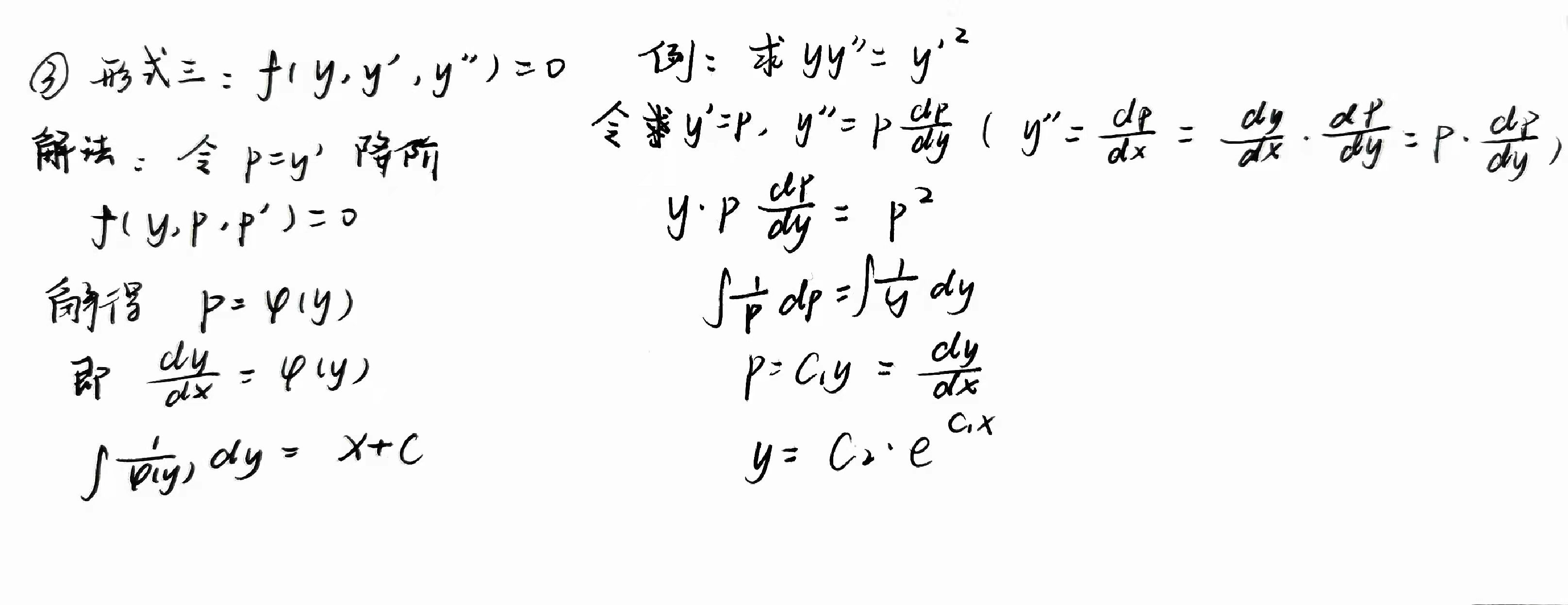二阶微分方程分为：

二阶常系数齐次线性微分方程；

二阶常系数非齐次线性微分方程；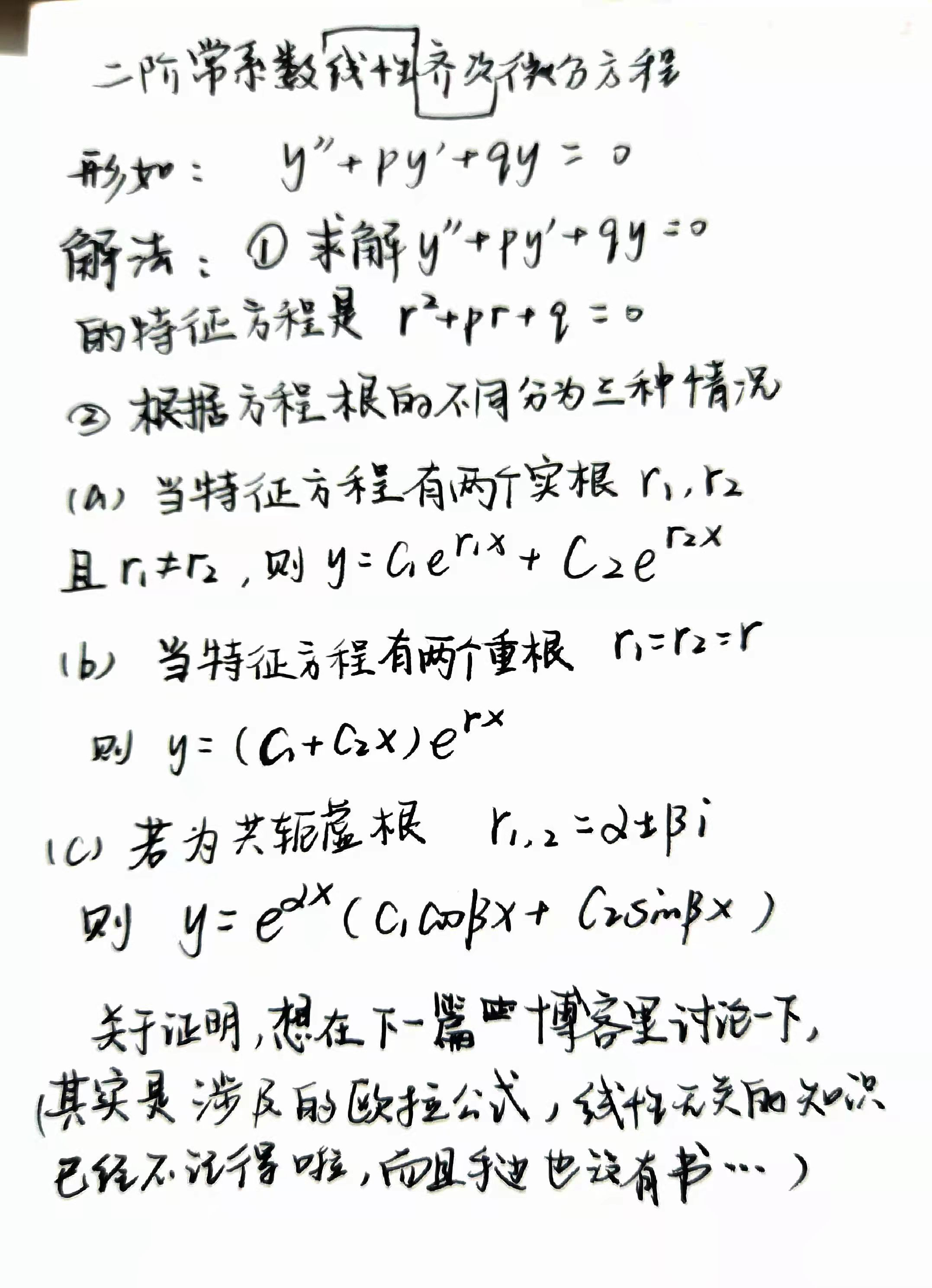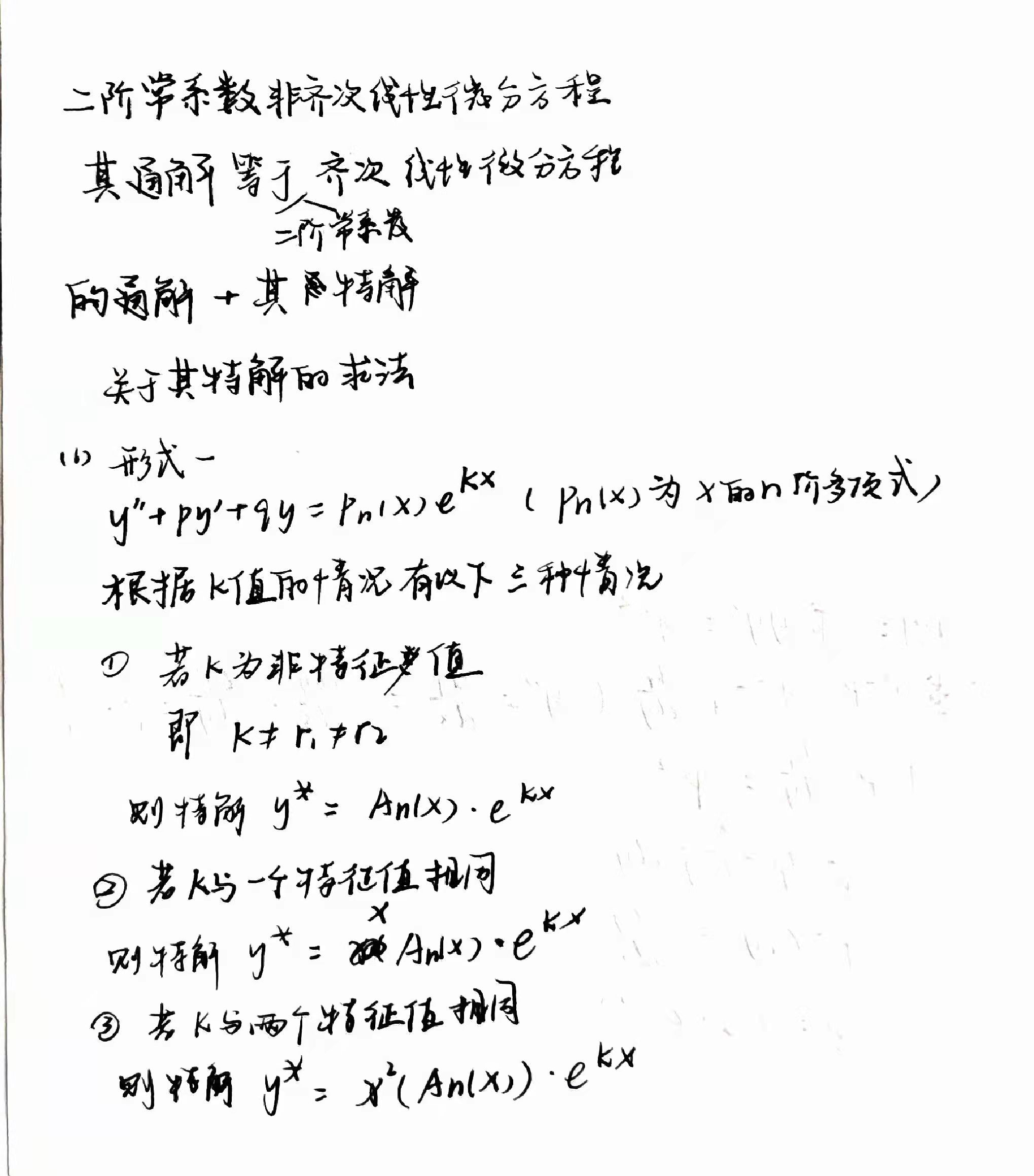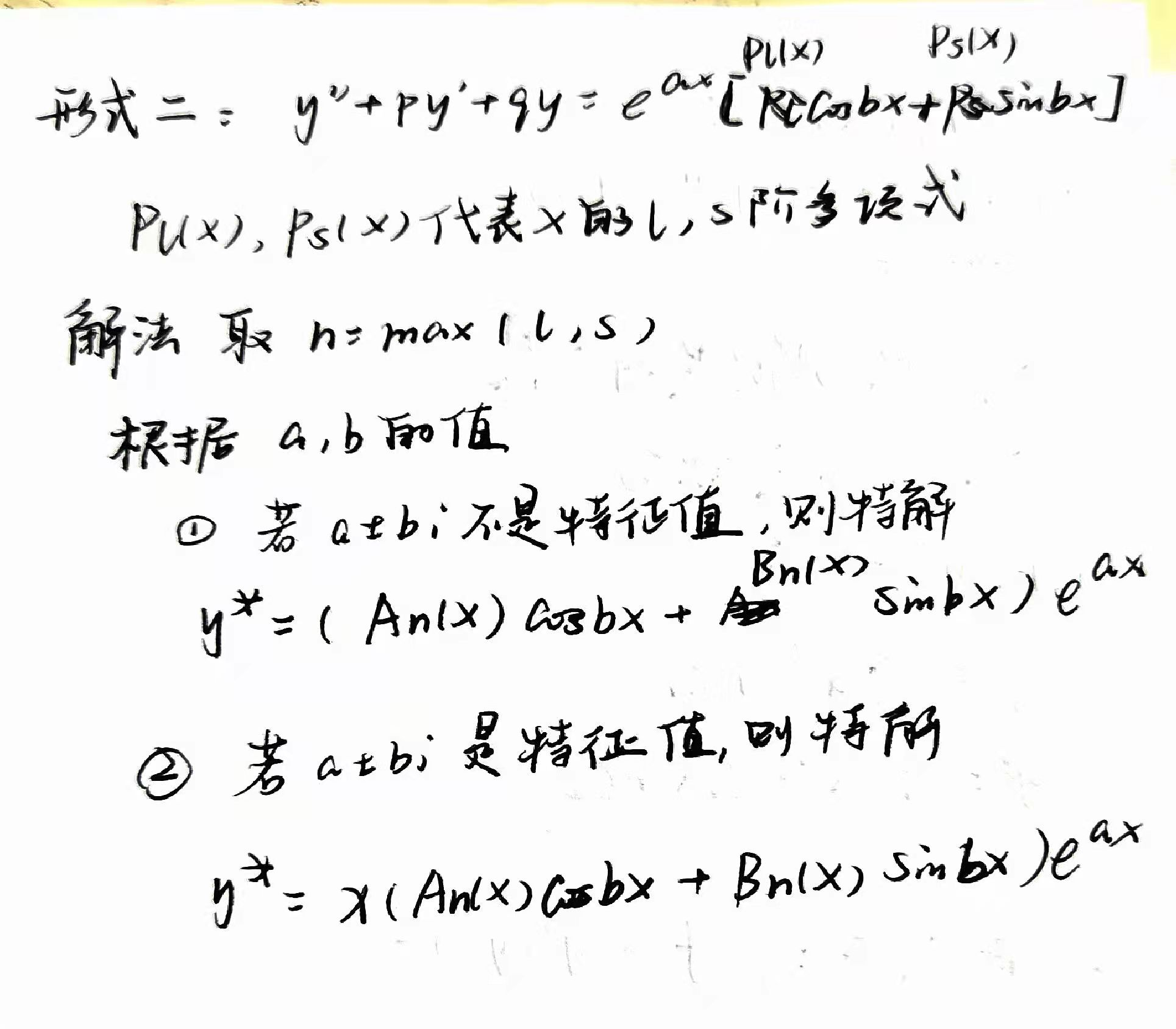那么，关于微分方程的通解就到此告一段落了。

第一次写博客，希望自己能坚持下去，我觉得写博客是一种很好的学习方式，写一篇博客没有想象中那么容易的。

展开全文学习
• 数学二阶线性微分方程理论及解法PPT学习教案.pptx
• 利用两类Riccati方程z′=z2 - a(x)z+ b( x)的求解公式,给出了两类二阶线性微分方程的通解,应用这些只与方程系数a(x)与b( x)相关的求解公式,求通解过程十分简捷.
• 一、线性微分方程的解的结构 1.1 二阶齐次线性方程 y′′+P(x)y′+Q(x)y=0(1) y''+P(x)y'+Q(x)y=0 \tag{1} y′′+P(x)y′+Q(x)y=0(1) 定理1：如果函数y1(x)y_1(x)y1​(x)与y2(x)y_2(x)y2​(x)是方程（1）的两个解，...

### 一、线性微分方程的解的结构

##### 1.1 二阶齐次线性方程

y ′ ′ + P ( x ) y ′ + Q ( x ) y = 0 (1) y''+P(x)y'+Q(x)y=0 \tag{1}

定理1：如果函数 y 1 ( x ) y_1(x) y 2 ( x ) y_2(x) 是方程（1）的两个解，那么
y = C 1 y 1 ( x ) + C 2 y 2 ( x ) (2) y=C_1y_1(x)+C_2y_2(x) \tag{2}
也是方程（1）的解，其中 C 1 , C 2 C_1,C_2 是任意常数。

解（2）从形式上看含有 C 1 C_1 C 2 C_2 两个任意常数，但它不一定是方程（1）的通解。那么在什么情况下（2）式才是方程（1）的通解呢？要解决这个问题，还得引入新概念，即函数组的线性相关与线性无关。

y 1 ( x ) , y 2 ( x ) , ⋅ ⋅ ⋅ , y n ( x ) y_1(x),y_2(x),···,y_n(x) 为定义在区间 I I 上的n个函数，如果存在n个不全为零的常数 k 1 , k 2 , ⋅ ⋅ ⋅ , k n k_1,k_2,···,k_n ，使得当 x ∈ I x\in I 时有恒等式
k 1 y 1 + k 2 y 2 + ⋅ ⋅ ⋅ + k n y n = 0 k_1y_1+k_2y_2+···+k_ny_n=0
成立，那么称这n个函数在区间I上线性相关；否则线性无关。

应用上述概念可知，对于两个函数的情形，它们线性相关与否，只要看它们的比是否为常数；如果比为常数，那么它们就线性相关；否则就线性无关。

定理2：如果 y 1 ( x ) y_1(x) y 2 ( x ) y_2(x) 是方程（1）的两个线性无关的特解，那么
y = C 1 y 1 ( x ) + C 2 y 2 ( x ) y=C_1y_1(x)+C_2y_2(x)
就是方程（1）的通解， C 1 , C 2 C_1,C_2 是任意常数。

推论：如果 y 1 ( x ) , y 2 ( x ) , ⋅ ⋅ ⋅ ， y n ( x ) y_1(x),y_2(x),···，y_n(x) 是n阶齐次线性方程
y ( n ) + a 1 ( x ) y ( n − 1 ) + ⋅ ⋅ ⋅ + a n − 1 ( x ) y ′ + a n ( x ) y = 0 y^{(n)}+a_1(x)y^{(n-1)}+···+a_{n-1}(x)y'+a_n(x)y=0
的n个线性无关的解，那么，此方程的通解为
y = C 1 y 1 ( x ) + C 2 y 2 ( x ) + ⋅ ⋅ ⋅ + C n y n ( x ) y=C_1y_1(x)+C_2y_2(x)+···+C_ny_n(x)
其中 C 1 , C 2 , ⋅ ⋅ ⋅ , C n C_1,C_2,···,C_n 为任意常数。

##### 1.2 二阶非齐次线性方程

一阶非齐次线性微分方程 的通解由两部分构成：一部分是对应的齐次方程的通解，另一部分是非齐次方程本身的一个特解。实际上，不仅一阶非齐次线性方程的通解具有这样的结构，而且二阶及更高阶的非齐次线性微分方程的通解也具有同样的结构。

定理3：设 y ∗ ( x ) y^*(x) 是二阶非齐次线性方程
y ′ ′ + P ( x ) y ′ + Q ( x ) y = f ( x ) (3) y''+P(x)y'+Q(x)y=f(x) \tag{3}
的一个特解。 Y ( x ) Y(x) 是与（3）对应的齐次方程（1）的通解，则
y = Y ( x ) + y ∗ ( x ) y=Y(x)+y^*(x)
是二阶非齐次线性方程（3）的通解。

由于对应的齐次方程（1）的通解 Y = C 1 y 1 + C 2 y 2 Y=C_1y_1+C_2y_2 中含有两个任意常数，所以 y = Y + y ∗ y=Y+y^* 中也含有两个任意常数，从而它就是二阶非齐次线性方程（3）的通解。

定理4：设非齐次线性方程（3）的右端 f ( x ) f(x) 是两个函数之和，即
y ′ ′ + P ( x ) y ′ + Q ( x ) y = f 1 ( x ) + f 2 ( x ) y''+P(x)y'+Q(x)y=f_1(x)+f_2(x)
y 1 ∗ ( x ) y_1^*(x) y 2 ∗ ( x ) y_2^*(x) 分别是方程
y ′ ′ + P ( x ) y ′ + Q ( x ) y = f 1 ( x ) y''+P(x)y'+Q(x)y=f_1(x)

y ′ ′ + P ( x ) y ′ + Q ( x ) y = f 2 ( x ) y''+P(x)y'+Q(x)y=f_2(x)
的特解，则 y 1 ∗ ( x ) + y 2 ∗ ( x ) y_1^*(x)+y_2^*(x) 就是原方程的特解。

这已订立通常称为线性微分方程的解的叠加原理

定理3和定理4也可推广到n阶非齐次线性方程。

### 二、 常数变易法

为解一阶非齐次线性方程，我们用了常数变易法。这方法的特点是：如果 C y 1 ( x ) Cy_1(x) 是齐次线性方程的通解，那么，可以利用变换 y = u y 1 ( x ) y=uy_1(x) （这变换是把齐次方程的通解中的任意常数C换成未知函数 u ( x ) u(x) 而得到的）去解非齐次线性方程。这一方法也适用于解高阶线性方程。下面就二阶线性方程来作讨论。

##### 2.1 已知齐次方程的两个解 y 1 ( x ) y_1(x) 和 y 2 ( x ) y_2(x)

如果已知齐次方程（1）的通解为
Y ( x ) = C 1 y 1 ( x ) + C 2 y 2 ( x ) Y(x)=C_1y_1(x)+C_2y_2(x)
那么，可以用如下的常数变易法去求非齐次方程（3）的通解。令
y = y 1 ( x ) v 1 + y 2 ( x ) v 2 (4) y=y_1(x)v_1+y_2(x)v_2 \tag{4}
要确定未知函数 v 1 ( x ) v_1(x) v 2 ( x ) v_2(x) 使（4）式所表示的函数满足非齐次方程（3）。为此对（4）式求导，得
y ′ = y 1 v 1 ′ + y 2 v 2 ′ + y 1 ′ v 1 + y 2 ′ v 2 y'=y_1v_1'+y_2v_2'+y_1'v_1+y_2'v_2
由于两个未知函数 v 1 , v 2 v_1,v_2 只需使（4）式所表示的函数满足一个关系式（3），所以可规定它们再满足一个关系式。从 y ′ y' 的上述表示可看出，为了使 y ′ ′ y'' 的表示式中不含 v 1 ′ ′ v_1'' v 2 ′ ′ v_2'' ，可设
y 1 v 1 ′ + y 2 v 2 ′ = 0 (5) y_1v_1'+y_2v_2'=0 \tag{5}
从而
y ′ = y 1 ′ v 1 + y 2 ′ v 2 y'=y_1'v_1+y_2'v_2
再求导，得
y ′ ′ = y 1 ′ v 1 ′ + y 2 ′ v 2 ′ + y 1 ′ ′ v 1 + y 2 ′ ′ v 2 y''=y_1'v_1'+y_2'v_2'+y_1''v_1+y_2''v_2
y , y ′ , y ′ ′ y,y',y'' 代入方程（3），化简得
y 1 ′ v 1 ′ + y 2 ′ v 2 ′ + ( y 1 ′ ′ + P y 1 ′ + Q y 1 ) v 1 + ( y 2 ′ ′ + P y 2 ′ + Q y 2 ) v 2 = f y'_1v'_1+y_2'v'_2+(y_1''+Py_1'+Qy_1)v_1+(y_2''+Py_2'+Qy_2)v_2=f
注意到 y 1 y_1 y 2 y_2 是齐次方程（1）的解，故上式即为
y 1 ′ v 1 ′ + y 2 ′ v 2 ′ = f (6) y_1'v_1'+y_2'v_2'=f \tag{6}
联立方程（5）与（6），在系数行列式
W = ∣ y 1 y 2 y 1 ′ y 2 ′ ∣ = y 1 y 2 ′ − y 1 ′ y 2 ≠ 0 W =\begin{vmatrix} y_1 & y_2 \\ y_1'& y_2' \end{vmatrix} =y_1y_2'-y_1'y_2 \neq 0
时，可解得
v 1 ′ = − y 2 f W ,   v 2 ′ = y 1 f W v_1'=-\frac{y_2 f}{W},\, v_2'=\frac{y_1f}{W}
对上两式积分（假定 f ( x ) f(x) 连续），得
v 1 = C 1 + ∫ ( − y 2 f W ) d x ,   v 2 = C 2 + ∫ y 1 f W d x v_1=C_1+\int(-\frac{y_2f}{W})dx,\, v_2=C_2+\int\frac{y_1f}{W}dx
于是得非齐次方程（3）的通解为
y = C 1 y 1 + C 2 y 2 − y 1 ∫ y 2 f W d x + y 2 ∫ y 1 f W d x y=C_1y_1+C_2y_2-y_1\int\frac{y_2f}{W}dx+y_2\int\frac{y_1f}{W}dx

##### 2.2 已知齐次方程的一个解 y 1 ( x ) y_1(x)

如果只知齐次方程（1）的一个不恒为零的解 y 1 ( x ) y_1(x) ，那么，利用变换 y = u y 1 ( x ) y=uy_1(x) ，可把非齐次方程（3）化为一阶线性方程。

事实上，把
y = y 1 u ,   y ′ = y 1 u ′ + u 1 ′ u ,   y ′ ′ = y 1 u ′ ′ + 2 y 1 ′ u ′ + y 1 ′ ′ u y=y_1u,\,y'=y_1u'+u_1'u,\, y''=y_1u''+2y_1'u'+y_1''u
代入方程（3），化简得
y 1 u ′ ′ + ( 2 y 1 ′ + P y 1 ) u ′ + ( y 1 ′ ′ + P y 1 ′ + Q y 1 ) u = f y_1u''+(2y_1'+Py_1)u'+(y_1''+Py_1'+Qy_1)u=f
由于 y 1 ′ ′ + P y 1 ′ + Q y 1 = 0 y_1''+Py_1'+Qy_1=0 ，故上式为
y 1 u ′ ′ + ( 2 y 1 ′ ′ + P y 1 ) u ′ = f y_1u''+(2y_1''+Py_1)u'=f
u ′ = z u'=z ，上式即化为一阶线性方程
y 1 z ′ + ( 2 y 1 ′ + P y 1 ) z = f (7) y_1z'+(2y_1'+Py_1)z=f \tag{7}
把方程（3）化为方程（7）以后，按一阶线性方程的解法，设求得方程（7）的通解为
z = C 2 Z ( x ) + z ∗ ( x ) z=C_2Z(x)+z^*(x)
积分得 u = C 1 + C 2 U ( x ) + u ∗ ( x ) u=C_1+C_2U(x)+u^*(x) （其中 U ′ ( x ) = Z ( x ) , u ∗ ’ ( x ) = z ∗ ( x ) U'(x)=Z(x),u^{*}{’}(x)=z^*(x) ），

上式两端乘 y 1 ( x ) y_1(x) ，便得方程（3）的通解
y = C 1 y 1 ( x ) + C 2 U ( x ) y 1 ( x ) + u ∗ ( x ) y 1 ( x ) y=C_1y_1(x)+C_2U(x)y_1(x)+u^*(x)y_1(x)
上式方法显然也适用于求齐次方程（1）的通解。

展开全文• 基于变分原理,将二阶线性微分方程的两点边值问题转化为等价的变分问题(即泛函极值问题),利用两点三次Hermite插值构造一个逼近可行函数的近似函数,从而将问题转化为一个多元单目标优化问题,最后运用粒子群优化算法...
•文档
•文档
• 啥是微分方程？普通方程我知道，就是x，y的关系式，比如y=2x，这算是一个方程。 微分方程就是，这个等式里不止有x，y两个元，还会有诸如，等存在。...一阶线性微分方程 即形如，左边是关于y的关系式...算法
• 一阶与二阶常系数线性微分方程及其解法PPT学习教案.pptx学习课件
• 通用的非线性微分方程四阶龙格库塔解法，取后四分之一稳定周期，可根据需要自己设置精度及初值，代入参数即可求解。
• 二阶线性微分方程就是指形如y′′+py′+q=0y&#x27;&#x27;+py&#x27;+q=0y′′+py′+q=0的微分方程，一种解法就是使用二阶微分方程的公式，这里介绍另一种方法。 对于二阶微分方程，如果找到两个线性...微分方程
• w摘 要本文主要研究二阶微分方程边值问题的数值解法。对线性边值问题，我们总结了两类常用的数值方法，即打靶法和有限差分方法，对每种方法都列出了详细的计算步骤和Matlab程序代码，通过具体的算例对这两类方法的...
• ## 二阶微分方程解法

千次阅读 2021-11-23 10:45:40算法
• 二阶线性微分方程的打靶法二阶线性微分方程的打靶法 计算思路 主要分为以下五步： 给定容许误差ε，迭代初始值γ1，对k=1,2，...做： (1)用四阶Runge-Kutta 方法求解初值问，得出u1之后取其在γk的值，从而...
• 微分方程的数值解法主要包括两大类：有限差分法和有限单元法。这里主要介绍有限单元法。 However，对于一个只学过微积分和矩阵论的工科生来说，要了解有限元法的数学原理还是有些困难，所以这里重点是介绍有限元法...计算数学
• 微分方程数值解法 一书中主要习题解答 及其习题拓展，纯手工录入，公式清晰，步骤清晰
• 二阶线性微分方程封闭统一显式解，于力，李峰，二阶微分方程，包括非线性微分方程，存在封闭统一显式解．封闭统一显式解指出，非线性微分方程必须在复变函数域中求解；方程解必
• 对于一般的二阶线性非齐次微分方程 我们先利用格林函数的思想来求解 固定每一个时刻，假定我们能求解 那么可以证明原方程的解就是 我们引入阶跃函数将上面等式上限变为正无穷 我们将上式改写为卷积...深度学习 矩阵
• 如何用matlab求解非线性微分方程组(基于龙格库塔的数值微分算法)？.docx...